Orginal Article

# 热致和磁致形状记忆合金循环变形和疲劳行为研究

1 西南交通大学牵引动力国家重点实验室, 成都 610031
2西南交通大学力学与工程学院应用力学与结构安全四川省重点实验室, 成都 610031
3 电子科技大学机电工程学院, 成都 611731

# Study on cyclic deformation and fatigue of thermal and magnetic shape memory alloys

KANG Guozheng12ɛ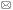, KAN Qianhua12, YU Chao12, SONG Di3

1 State Key Laboratory of Traction Power, Southwest Jiaotong University, Chengdu 610031, China
2 Sichuan Provincial Key Laboratory of Applied Mechanics and Structure Safety, School of Mechanics and Engineering, Southwest Jiaotong University, Chengdu 610031, China
3 School of Mechatronics Engineering, University of Electronic Science and Technology of China, Chengdu 611731, ChinaAbstract

Shape memory alloys (SMAs), including the thermal and magnetic ones, have attracted wide attention from scholars and engineers, and have been successfully employed in many engineering applications due to their unique super-elasticity and shape memory effects. To further prompt the application fields of SMAs, the progresses in the microscopic and macroscopic experimental observations and theoretical studies on their thermo-mechanical and magneto-mechanical coupled cyclic deformation and fatigue failure are reviewed herein. The latest achievements on the thermo-mechanical coupled cyclic deformation and fatigue failure of the thermal (i.e., temperature-induced) SMAs including NiTi and high-temperature NiTiX ones are summarized. In addition, the research status on the magneto-mechanical coupled cyclic deformation and fatigue failure of the magnetic SMAs including NiMnGa ones are reviewed. Last, a brief summary and a prospect of future topics are provided.

Keywords： shape memory alloy ; cyclic deformation ; fatigue failure ; micro- and macro-scales ; experimental observation ; theoretical model

0

KANG Guozheng, KAN Qianhua, YU Chao, SONG Di. Study on cyclic deformation and fatigue of thermal and magnetic shape memory alloys[J]. Advances in Mechanics, 2018, 48(0): 1802- https://doi.org/10.6052/1000-0992-17-008

## 2 热致形状记忆合金的热--力耦合循环变形行为

### 2.1 NiTi形状记忆合金的循环变形行为

2.1.1 实验研究

2.1.1.1 超弹性NiTi形状记忆合金的率相关循环变形行为

(1) 宏观变形行为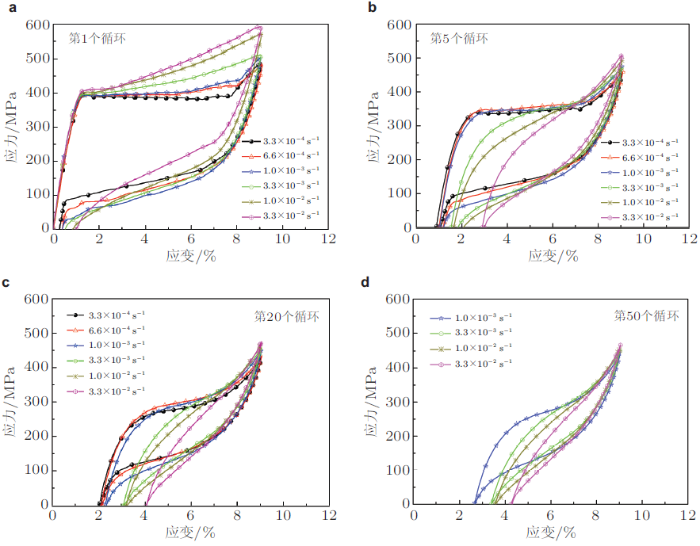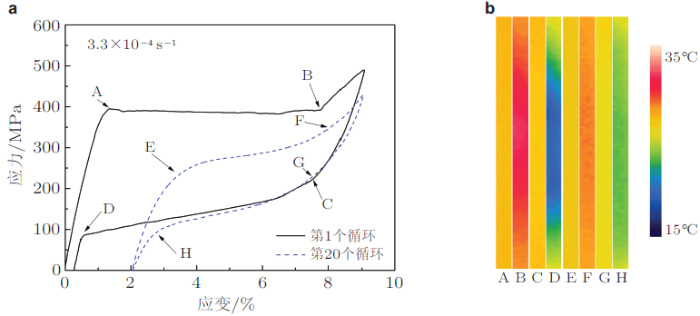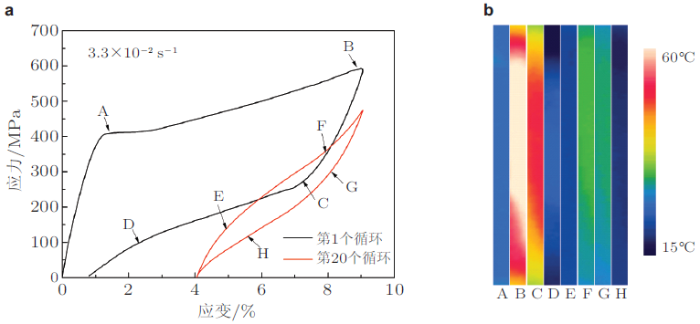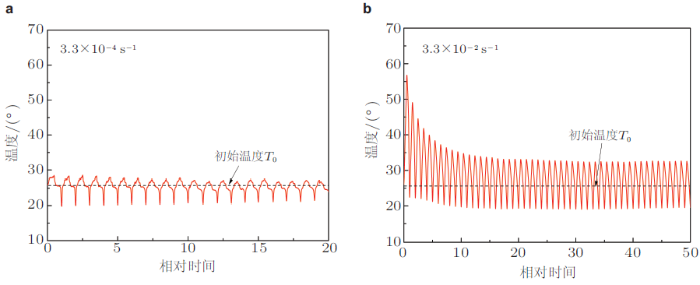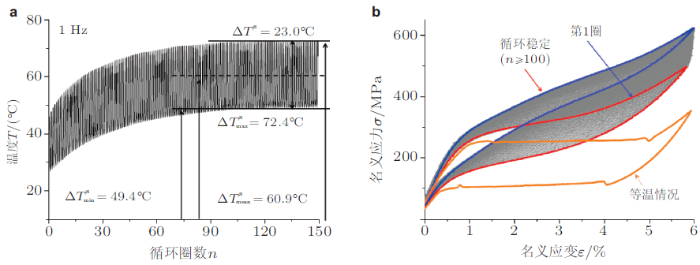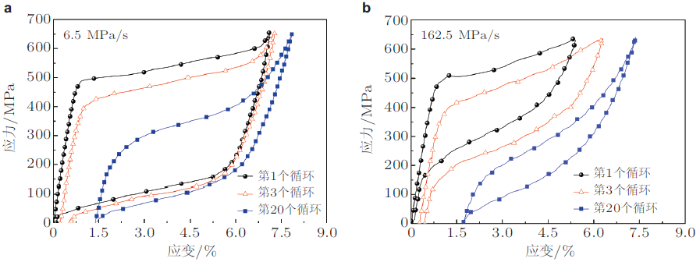(2) 相变图案及其演化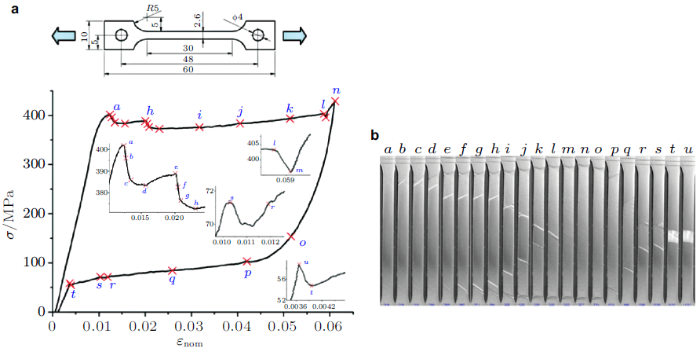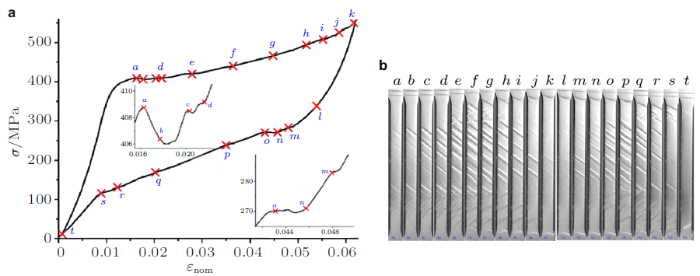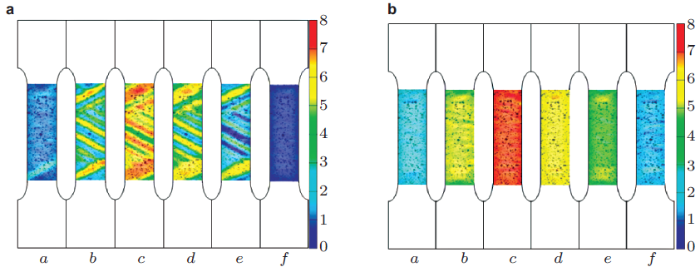Xie等(2016b)进一步分别讨论了在应变控制和应力控制拉伸--卸载循环变形过程中相变图案的形成和演化对加载速率的依赖性.研究表明: (1)相变条带的数目随加载速率的增大而单调增大; (2)在加载速率较低时, 随循环次数的增大, 变形逐渐由明显的局部化特征过渡到均匀变形, 残余马氏体条带不明显; (3)在加载速率较大时, 在循环稳定时将会有明显的残余马氏体条带产生, 这是因为加载速率较快时, 相变棘轮行为更为显著, 增加的位错密度将对马氏体逆相变产生显著的抑制作用的缘故; (4)在应力控制的循环拉伸--卸载加载的稳定变形阶段, 观察到的应变和温度场要比应变控制下的更为均匀.材料内部的相变热生成和与外界的热交换之间的相互竞争是相变图案率相关性的根本原因, 而在稳定的循环变形阶段这两种因素的竞争达到一个动态的平衡.

2.1.1.2 形状记忆效应NiTi合金的率相关热--力耦合循环变形行为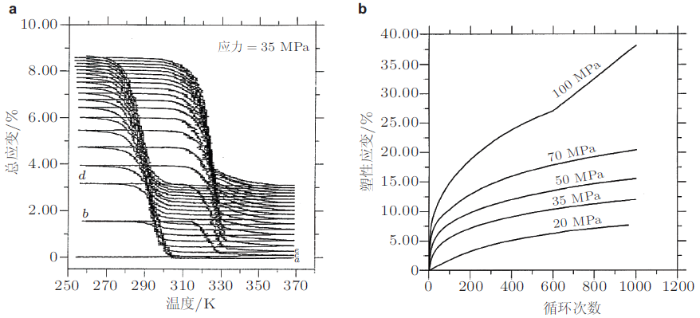2.1.2 微观实验观察

Pelton 等(2011, 2012)采用透射电子显微镜(TEM)对NiTi形状记忆合金在温度循环过程中的微观结构演变进行了观察, 结果发现位错密度会随着温度循环次数的增大而逐渐增大, 如图13所示, 并且累积的位错会影响材料的温度和力学响应.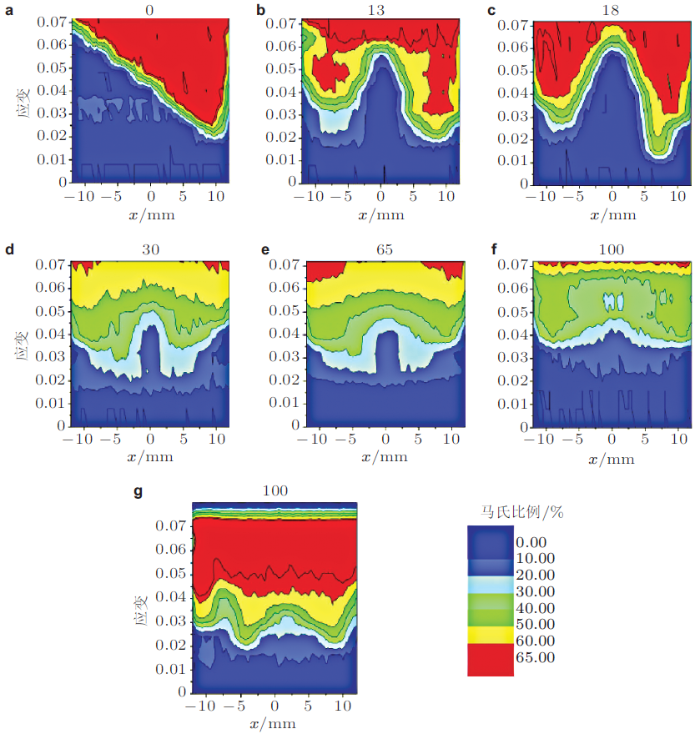Tadayyon等(2017)通过TEM观察研究了初始相为马氏体的NiTi形状记忆合金在拉伸载荷作用下的变形行为和相关的微结构演变, 如图16所示. 结果表明: 初始拉伸阶段首先发生孪晶马氏体的弹性变形, 然后发生马氏体解孪并进一步产生位错, 而位错的移动则会促进解孪和孪生变形; 随着变形的增大, 位错之间的相互约束增强, 最后发生剪切带和堆垛层错的滑移. Tadayyon等(2017)指出位错运动和孪生变形可以细化晶粒, 导致合金强度提高以及延展性增强, 而堆垛层错进一步使得材料发生加工硬化. 这些研究结果表明, NiTi形状记忆合金发生马氏体重定向时也会产生大量的位错缺陷, 且位错缺陷甚至会演变成堆垛层错, 进一步影响该合金的性能.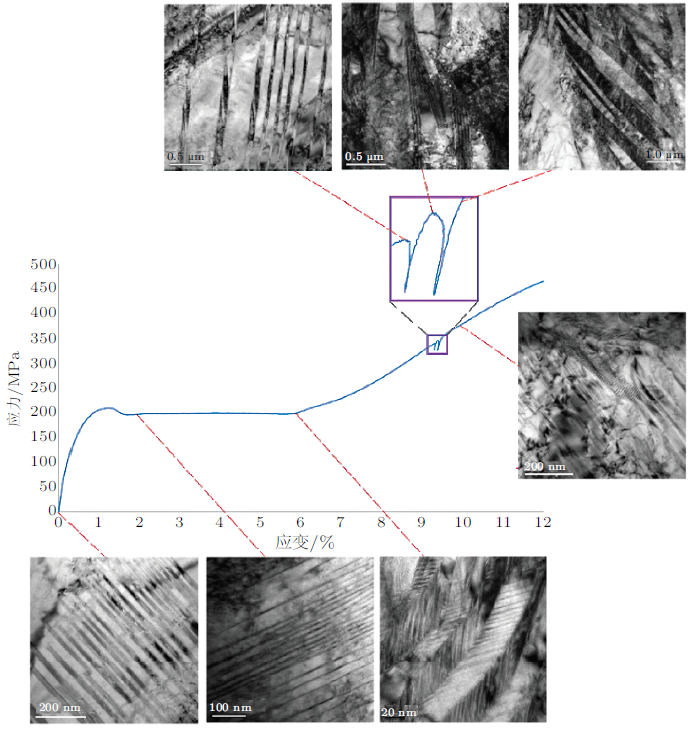2.1.3 微/纳尺度的数值模拟

Cisse等(2016)在对形状记忆合金的本构模型和模拟技术的综述过程中对相关的分子动力学模拟和相场分析进行了简要评述, 但并没有涉及到一些具体细节和重要结果, 并且对形状记忆合金在微纳米尺度下的热--力耦合循环变形行为数值模拟讨论较少.

2.1.3.1 相场模拟

2.1.1.1节中讨论了相变图案及其演化结果, NiTi形状记忆合金的相变过程是一个局部形核、逐渐长大的变化过程, 而采用一般的本构模型是无法模拟形状记忆合金这种局部相变行为的演化.但相场模拟可以通过系统总能量最小化得到材料微结构演变, 在得到了材料系统的自由能泛函之后, 根据相场动力学方程进行序参量的求解.相场分析方法由于具有处理复杂的几何和拓扑变化的灵活性特点, 已广泛应用于模拟相变过程中微观结构的演变.不同序参量满足的动力学方程不同, 根据序参数的保守和非保守性, 描述材料微结构演变的相场动力学方程有两种简单的近似形式:

(1) Cahn-Hilliard模型 (Cahn & Hilliard 1958, 1959), 这种模型的相由保守的场变量唯一决定; (2) Allen-Cahn模型 (Cahn {\rm&} Allen 1977, Allen & Cahn 1979), 这种模型的相无法由浓度、温度和压强等唯一决定, 而需要引入一个或多个额外的非保守场变量即序参量来决定局部相. 目前, 大部分的相场模拟是基于Allen-Cahn模型及其改进模型开展的.Allen-Cahn模型的动力学方程通常也称之为时间相关Ginzburg-Landau(TDGL)方程, 求解该动力学方程的数值解法有很多, 最常见的是基于统一空间网格和精确时间步长的简单二阶有限差分法 (Fan & Chen 1997). 从已有研究中可归纳出相场模拟的4个步骤: (1)为研究材料系统确定合适的序参数; (2)将局部能量密度表示为所选序参数的函数, 并得出材料系统的自由能泛函, 这些函数必须正确描述系统的对称性和热力学性质; (3)由自由能泛函得到相场动力学方程, 并将从实验数据或基础计算中得到的唯象系数带入动力学方程; (4)确定正确的初始和边界条件并选择适当的方法求解动力学方程.

Paranjape 等(2016)基于相场理论的有限元模拟方法, 采用晶体塑性理论模拟形状记忆合金单晶温度诱发马氏体相变和塑性滑移之间的交互作用, 如图17所示. 模拟结果表明: (1)奥氏体和马氏体界面的局部变形不协调诱发的高应力容易导致奥氏体发生塑性滑移; (2)马氏体和马氏体界面附近材料点的等效塑性应变较大; (3)冷却阶段塑性滑移量的增加比升温阶段要慢; (4)塑性滑移较小区域促进马氏体相变;(5)塑性滑移较大区域处马氏体相变受阻.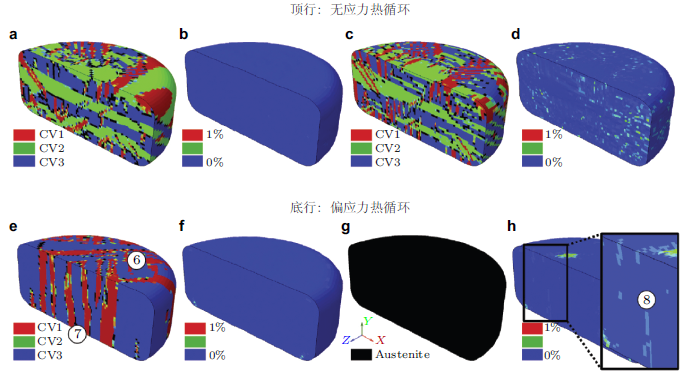Levitas 和 Javanakht (2012)引入位错序参量, 采用有限元法研究了奥氏体相和奥氏体--马氏体界面处位错的形核和扩展过程.之后, Levitas 和 Javanakht (2013)同时引入相变序参量和位错序参量, 采用有限元方法研究了纳米尺度平面应变大变形情况下马氏体(单个变体)相变、位错对奥氏体--马氏体界面扩展的影响、温度诱发马氏体相变时马氏体片的演化和应力诱发马氏体相变时微结构的演化.同时, Levitas 和 Javanbakht(2015)还详细介绍了纳米尺度大变形情况下马氏体(多个变体)相变、孪生和位错之间交互作用理论的推导和依据. 然后, Javanbakht和 Levitas (2015)采用有限元方法对该模型进行了数值实现, 进而研究了纳米尺度应力诱发双晶马氏体相变与位错之间的交互作用, 发现位错引起的应力集中可以促进马氏体形核, 而位错引起的应力释放又会阻碍马氏体相变. 此外, 他们还研究了温度诱发马氏体相变时马氏体片在双晶之间的扩展与位错之间的交互作用.

2.1.3.2 分子动力学模拟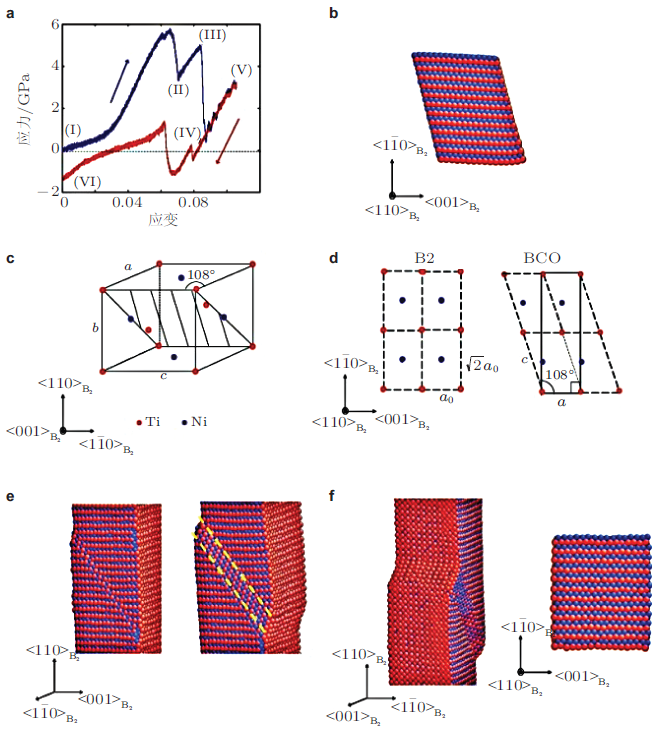Ko等(2017)则采用考虑第二近邻的MEAM势在奥氏体相变结束温度以上模拟了不同晶粒尺寸(应变率为5 $$\times$$ 10$$^{8}$$ s$$^{ - 1})$$和不同应变率 (晶粒尺寸为8nm)下NiTi形状记忆合金的超弹性行为, 分子动力学模拟结果表明: (1)加/卸载过程中的相变应力随着晶粒尺寸的减小而增加, 如图19所示; (2)在没有内部缺陷的模型中再现了实验中观察到的温度和应力诱导马氏体相变, 证实了内部缺陷贡献机制不是马氏体相变的主要诱发机制.基于分子动力学模拟结果, Ko等(2017)提出了相变诱发的应变贡献机制并成功地解释了马氏体相变和逆相变中的非对称形核和生长行为, 如图20所示(图中原子的颜色根据CNA模式进行标记, 在每幅截图中蓝色的原子对应B2奥氏体相, 红色的原子对应B19'马氏体相, 灰色的原子对应晶界和域边界).(3)超弹性NiTi形状记忆合金在拉伸过程的应力--应变响应强烈依赖于加载速率, 加载速率越大, 相变硬化程度越高, 进而相同应变值下对应的应力值越高; 同时, 相变开始应力也随加载速率的增大而升高, 如图21所示.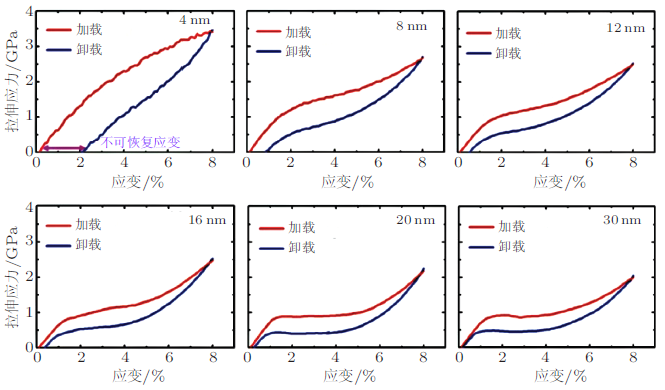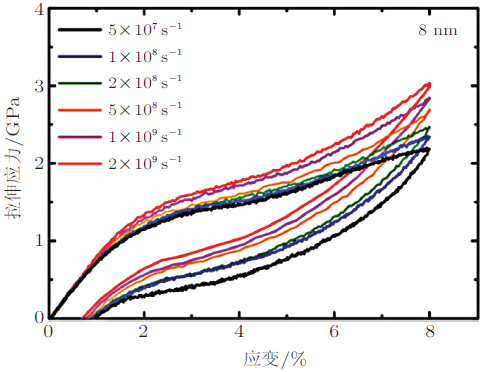Mirzaeifar 等(2014)采用Zhong等(2012)修正的NiTi形状记忆合金原子间相互作用势(EAM势)对温度和应力驱动下NiTi形状记忆合金纳米柱的相变过程进行了分子动力学模拟, 结果发现: (1)减小NiTi合金纳米柱的表面能可以使B2相奥氏体转变成B19相马氏体, 模型在弛豫后处于B19相(B19相中构成变体2的原子以红色表示), 如图22所示.(2)在轴向加载过程中变体2(V2)重定向成变体1(V1)：当应变加载到0.02时, 变体2(V2)中三个区域沿着大约$$\pm 45^\circ$$的方向转变成变体1(V1); 随后, 更多的变体2(V2)转变成变体1(V1); 在应变加载到0.1时, 变体2(V2)几乎全部转变成变体1(V1), 如图22(b)所示. (3)NiTi形状记忆合金纳米柱在三个不同温度下马氏体重定向过程的应力--应变曲线中明显可以看出重取向应力随着温度的升高而升高, 如图23所示.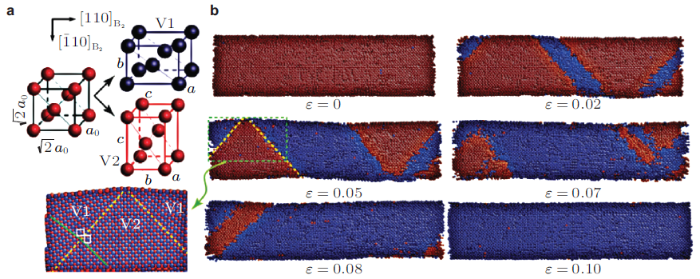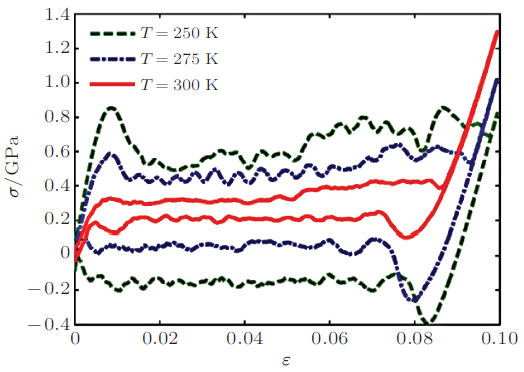Mutter 和 Nielaba(2013)采用EAM势对包含不同方向变体的马氏体相NiTi形状记忆合金纳米柱的拉伸--卸载和升温过程进行了原子模拟, 研究了纳米尺度下NiTi形状记忆合金的形状记忆效应, 在1K下具有5种不同Ni原子含量的NiTi形状记忆合金纳米柱的应力--应变曲线如图24所示, 结果表明NiTi形状记忆合金纳米柱在拉伸过程中多取向变体构型转变成单个变体构型, 卸载后NiTi合金纳米柱存在残余应变, 升温后并没有发现奥氏体产生.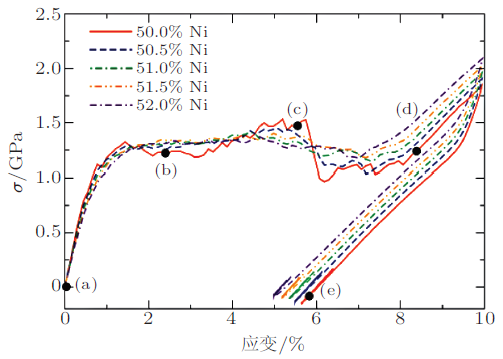Wang 等(2017)采用EAM势, 通过分子动力学模拟研究了循环压缩--卸载过程中NiTi形状记忆合金纳米柱的超弹性行为, 发现位错和孪生对原子模型的物理结构和力学响应的改变、累积不可逆应变和超弹性行为的衰减有着重要影响.图25给出了NiTi形状记忆合金纳米柱在400 K下峰值应变为7.46%时的单轴循环压缩--卸载模拟结果, 可见在循环变形过程中会产生明显的残余变形以及超弹性劣化现象, 循环7周以后即达到稳定状态, 超弹性滞回环消失.2.1.4 热--力耦合本构理论

Yu 等 (2014a)在小变形框架下, 将材料点总的应变张量$$\pmb \varepsilon$$分解为四个部分, 即弹性应变张量$$\pmb \varepsilon ^{\rm e}$$、热膨胀应变张量$$\pmb \varepsilon ^{\rm T}$$、相变应变张量$$\pmb\varepsilon ^{\rm tr}$$和相变诱发塑性应变张量$$\pmb \varepsilon^{\rm p}$$, 即$\pmb \varepsilon = \pmb \varepsilon ^{\rm e} +\pmb \varepsilon ^{\rm T} + \pmb \varepsilon ^{\rm tr} + \pmb\varepsilon ^{\rm p}\ \ (1)$热膨胀应变和温度的关系为$\pmb \varepsilon ^{\rm T} = \pmb \alpha(T - T_{\rm r} )\ \ (2)$其中, $$\pmb \alpha$$, $$T$$, $$T_{\rm r}$$分别是二阶热膨胀张量、当前温度和参考温度.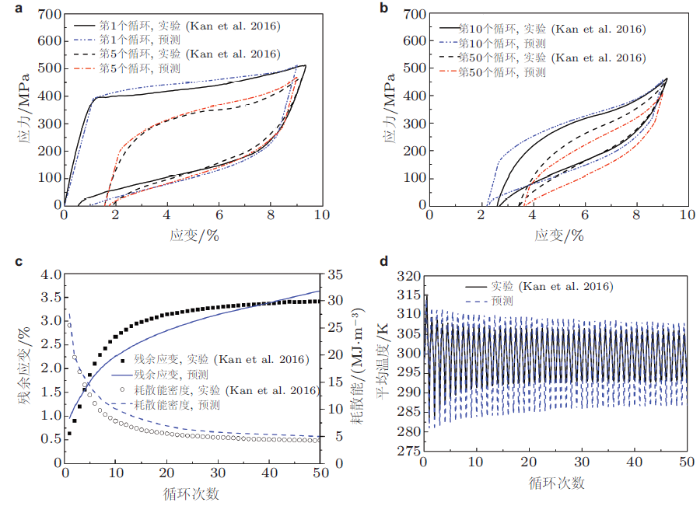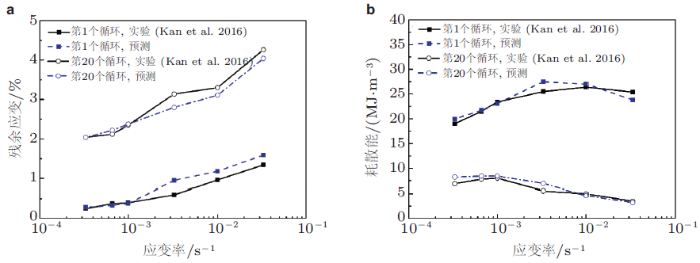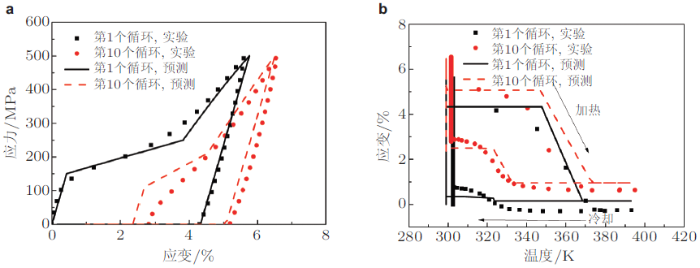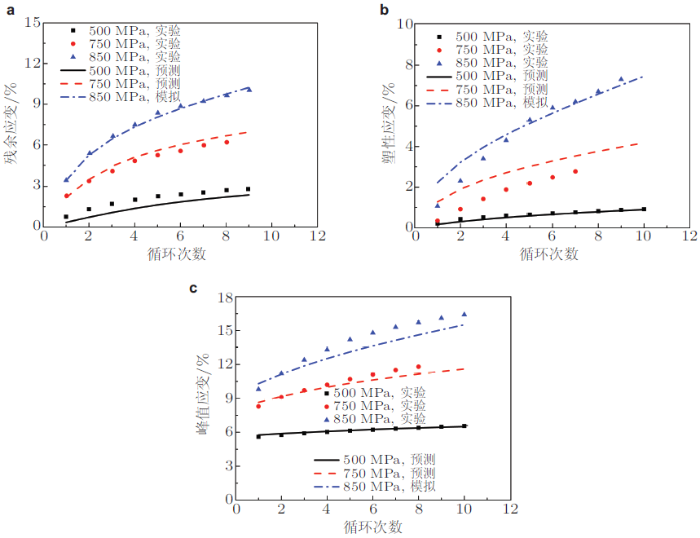### 2.2 高温NiTiX形状记忆合金的循环变形行为

2.2.1 实验研究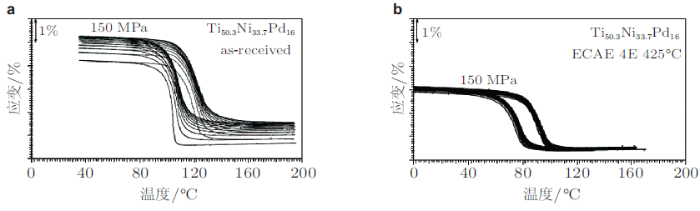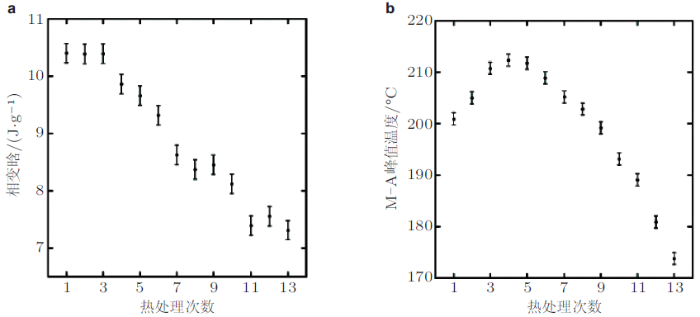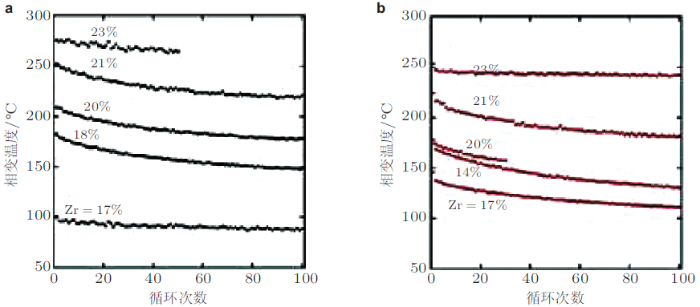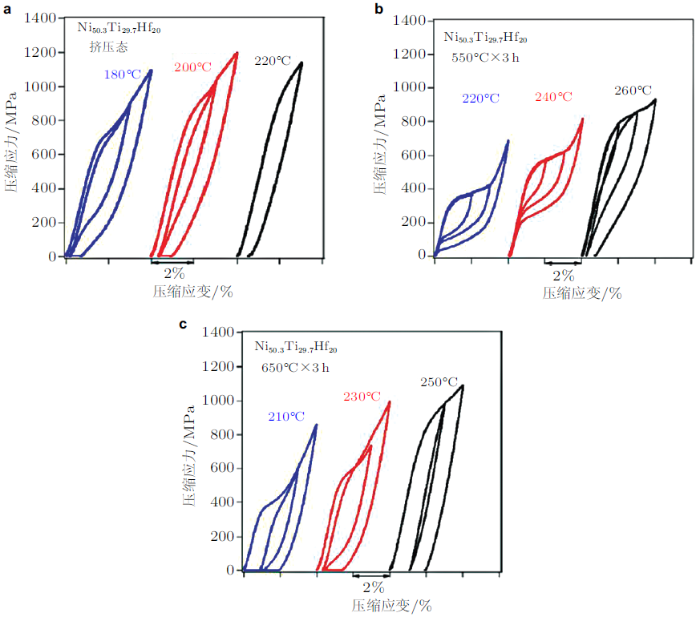2.2.2 微观实验研究

Kockar 等(2006, 2010)对供货态和挤压态NiTiPd和NiTiHf高温形状记忆合金的马氏体形貌分别进行了对比, 发现经过等通道转角挤压的高温形状记忆合金中马氏体尺寸比供货态有大幅度的减小, 进而材料的力学性能则有大幅度的提高. Meng 等(2002)对NiTiHf高温形状记忆合金在单次加载下的应力诱发马氏体形貌进行了观察, 发现: 在548 K下施加8%的应变时, 形成的马氏体具有方向性, 且边界较为清晰; 而应变增大到16%的时候, 马氏体的边界则变得模糊不清; 同时, 当实验温度升高到573 K时, 施加8%的应变即出现马氏体边界不清的情况, 与较低温度时的剧烈变形情况相似, 见图34.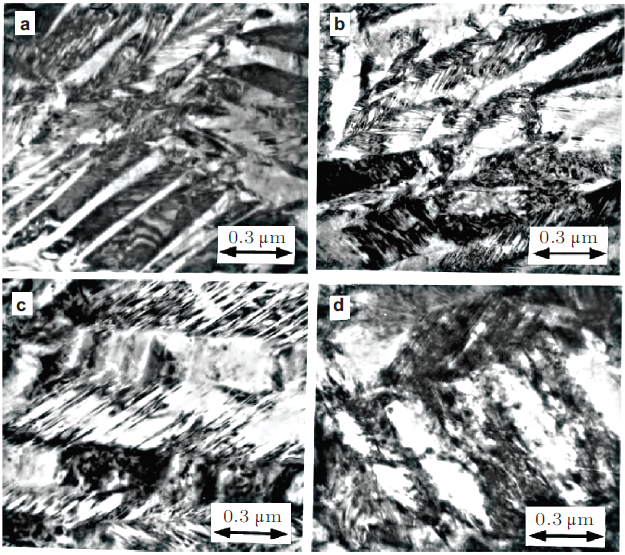Kumar & Lagoudas(2010)利用TEM对蠕变以及温度循环后的NiTiPd高温形状记忆合金的马氏体形貌分别进行了观察, 实验表明: 供货态材料中的马氏体以\{1 \ 1 \ 1\}取向的第一类孪晶构成, 见图35(a); 而蠕变加载下的马氏体则主要由\{0 \ 1 \ 1\}取向的复合孪晶和\{1 \ 1 \ 1\}取向的第一类孪晶同时组成, 并且复合孪晶在材料的微结构中占主导地位(图35(b)), 这些复合孪晶常出现在材料中的沉淀及缺陷周围; 同时, 蠕变加载中的位错攀移会导致位错在材料中出现随机分布的形式, 在取向不占优的滑移平面, 这些位错也会引起应力集中, 导致材料中复合孪晶的形成; 在温度循环中形成的马氏体尺寸比蠕变情况下更大, 且边界更为清晰, 其中\{1 \ 1 \ 1\}取向的孪晶在温度循环加载时占主导地位; 将试样加热到更高的温度, 材料中的马氏体恢复为奥氏体的比率也会相应增大, 见图36.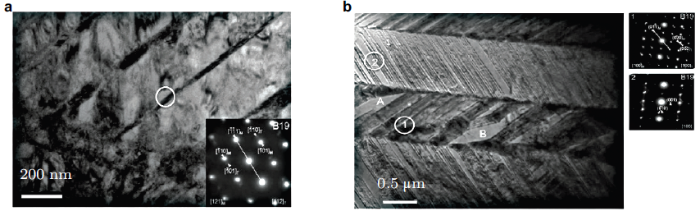2.2.3 本构理论

(1)相变变形

$\pmb\varLambda^{\rm t}_{\rm rev}=\dfrac{\pmb\varepsilon^{t-r}}{\xi^{r}},\qquad \dot{\xi}<0 \ \ {\rm (25b)}$其中, $$\pmb\sigma'$$为偏应力张量, $$\bar{\sigma}$$为von-Mises等效应力, $$H^{\rm cur}$$为最大相变应变, $$\pmb\varepsilon^{t-r}$$为逆相变开始时的相变应变张量, $$\xi^{\rm r}$$为逆相变时的马氏体体积分数. 对于正相变过程, 相变面$$\varPhi_{\rm fwd}^{\rm t}$$定义为$\varPhi_{\rm fwd}^{\rm t}=\pi_{\rm fwd}^{\rm t}-Y_{\rm fwd}^{\rm t}\ \ (26)$ 其中, $$\pi_{\rm fwd}^{\rm t}$$为正相变时的热力学驱动力, $$Y_{\rm fwd}^{\rm t}$$为临界能.$$Y_{\rm fwd}^{\rm t}$$可以写为(Hartl et al. 2010)$Y_{\rm fwd}^{\rm t}(\pmb\sigma)=Y_0^{\rm t}+D\pmb\sigma:\left(\pmb\varLambda_{\rm fwd}^{\rm t}+\pmb\varLambda_{\rm fwd}^{\rm tp}\right) \ \ (27)$ 其中, $$D$$为描述热力学驱动力对应力依赖程度的参数, $$Y_0^{\rm t}$$为常数.

(2)相变诱发塑性变形

(3)黏塑性变形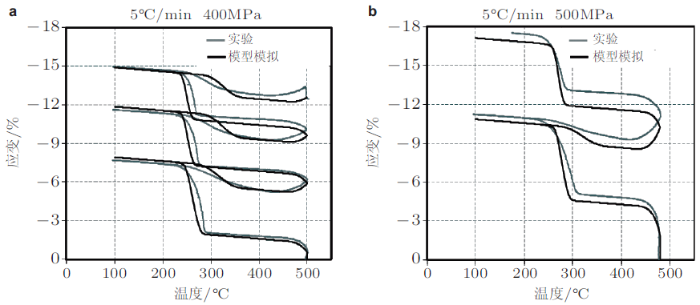## 3 温度诱发形状记忆合金的热--力耦合疲劳失效行为

### 3.1 NiTi形状记忆合金的疲劳失效行为

3.1.1 失效机理的微观实验研究

(4)超弹性NiTi形状记忆合金微管的多轴疲劳失效过程中在裂纹萌生阶段出现的较高程度的损伤以及特有的多方向、多平面微裂纹扩展模式使得其疲劳寿命远低于相同加载水平下的单轴疲劳寿命.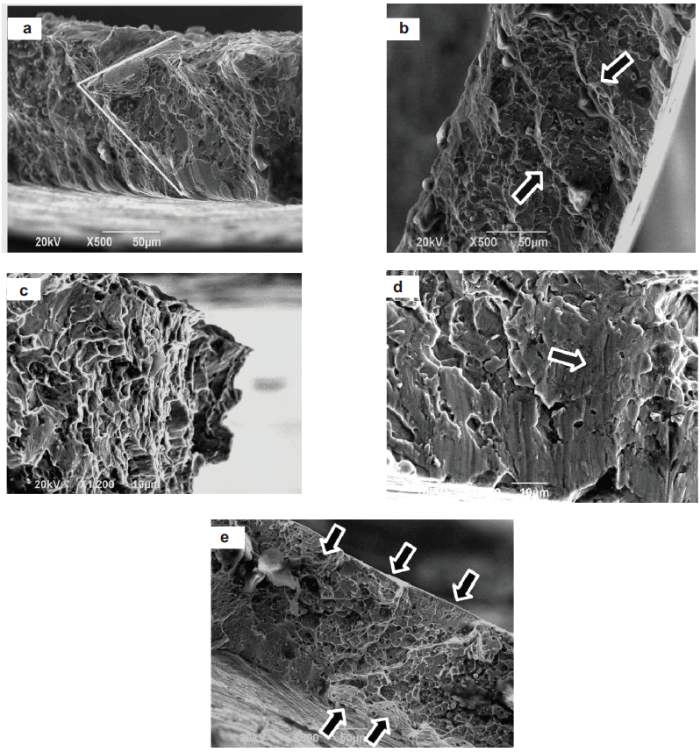Frotscher等(2008)利用原位SEM观测了NiTi形状记忆合金菱形结构的疲劳断裂过程.研究表明, 表面划痕以及缺陷等应力集中处易于出现裂纹萌生, 但实验中也发现一些表面缺陷处在循环过程中并不会造成裂纹萌生. 图40描述了在结构循环至第34周, 应变为24%时微裂纹的扩展情况.此时当应变仅增加0.06%时, 对比图40(a)与图40(b), 可见表面微裂纹尺寸明显增大. 继续施加应力时, 则材料迅速破坏.这也说明了裂纹萌生阶段占疲劳寿命的比例较大, 宏观裂纹一旦出现, 材料则在短时间内即发生破坏.3.1.2 半经验型疲劳失效模型

Zhang 等(2016)在室温下对NiTi合金丝进行了不同应变频率下的疲劳实验, 基于实验结果, 将非弹性耗散能分为两部分: 一部分通过热传导和热对流释放到环境中, 另一部分则储存于材料内部促进应力诱发马氏体相变的晶体缺陷.随加载速率的变化, 这两部分非弹性耗散能的比例发生变化, 由于释放到环境中的非弹性耗散能不会引起裂纹的萌生和扩展, 因此只考虑非弹性耗散能中储存于材料内部的部分达到一定临界值时, 裂纹开始萌生和扩展. 当加载速率越高, 材料内部储存的能量越大, 从而引起更低的寿命. Zhang等(2016)在此基础上发展了如下基于耗散能的疲劳准则$W_{\rm sat} = \gamma \dot {\varepsilon }^{ - \eta}N_{\rm f}^\beta \ \ (36)$其中, $$\dot {\varepsilon }$$为应变率, $$\gamma$$, $$\eta$$和$$\beta$$为材料参数, 可通过实验数据的拟合得到.该模型能够较合理的预测NiTi合金丝在不同应变率下的疲劳失效寿命.

Zhang 等(2017)为了进一步考虑加载模式和载荷比对NiTi合金丝疲劳寿命的影响, 提出了基于应变能的疲劳寿命预测模型$e_0 = \alpha N_{\rm f}^\beta\ \ (37)$其中, $$e_{0}$$为应变能, $$\alpha$$和$$\beta$$为材料参数.该模型能够较为合理的预测应变控制不同应变幅值和应力控制不同应力幅值下的疲劳失效寿命.

3.1.3 基于损伤演变的寿命预测模型

Song等(2015)首先在超弹性NiTi形状记忆合金单轴应力控制疲劳实验的基础上, 将损伤变量$$D$$定义为一定循环次数$$N$$下的累积耗散能密度与材料疲劳失效时$$(N_{\rm f})$$总的累积耗散能密度之比, 即$D = \dfrac{\sum\limits_{i = 1}^N {W_i }}{\sum\limits_{i = 1}^{N_{\rm f} } {W_i } } \ \ (38)$其中, $$W_{i}$$为一个循环次数内的耗散能密度.该损伤变量的定义考虑了稳定循环达到之前的循环变形行为对总的疲劳损伤的影响.

(1)由位错滑移引起的微裂纹萌生造成的损伤$$D_{\rm ini}$$$D_{\rm ini} = g_1 \cdot \int_0^{N_{\rm sat} } {W_N } {\rm d}N,\qquad N \leq N_{\rm sat} \ \ (39)$

(2)微裂纹稳态扩展造成的损伤$$D_{\rm pro}$$$D_{\rm pro} = g_2 \cdot \left( {N - N_{\rm sat} - 1} \right),\qquad N >N_{\rm sat} \ \ (40)$

(3)由循环马氏体相变和相变诱发塑性造成的损伤$$D_{\rm trans}$$$D_{\rm trans} = g_3 \cdot \left[ {\int_0^{N_{\rm sat} }{W_N {\rm d}N + (N - N_{\rm sat} - 1)} \cdot W_{\rm sat} } \right]\ \ (41)$其中$g_1 = b_1 \cdot \left( {\dfrac{\sigma _{\rm peak} - \sigma_{\rm AM}^{\rm s} }{\sigma _{\rm MA}^{\rm s} }} \right)^{n_1 }\ \ (42)$$g_2 = a_1 \cdot \dfrac{\sigma _{\rm peak} ^2 + \left[{\sigma _{\rm valley} \cdot H(\sigma _{\rm valley} )}\right]^2}{W_{\rm sat} } \ \ (43)$$g_3 = b_2 \cdot \left\{ {\left[ {\dfrac{F_1 (\sigma_{\rm peak} ) + F_2 (\sigma _{\rm peak} ,\sigma _{\rm valley})}{\sigma _{\rm AM}^{\rm f} - \sigma _{\rm AM}^{\rm s} + \sigma_{\rm MA}^{\rm s} - \sigma _{\rm MA}^{\rm f} }} \right]^{n_2 } +\left[ {\dfrac{F_1 (\sigma _{\rm peak} ) + F_2 (\sigma _{\rm peak},\sigma _{\rm valley} )}{\sigma _{\rm AM}^{\rm f} - \sigma _{\rm AM}^{\rm s} + \sigma _{\rm MA}^{\rm s} - \sigma _{\rm MA}^{\rm f}}} \right]^{\frac{1}{n_2 }}} \right\} \ \ (44)$$F_1 = (\sigma _{\rm peak} - \sigma _{\rm AM}^{\rm s} ) \cdot H(\sigma _{\rm peak} - \sigma _{\rm AM}^{\rm s} ) + (\sigma _{\rm AM}^{\rm f} - \sigma _{\rm peak} ) \cdot H(\sigma _{\rm peak} -\sigma _{\rm AM}^{\rm f} ) \ \ (45)$$F_2 = [(\sigma _{\rm MA}^{\rm s} - \sigma _{\rm valley} ) \cdot H(\sigma _{\rm MA}^{\rm f} - \sigma _{\rm valley} ) +$$(\sigma_{\rm valley} - \sigma _{\rm MA}^{\rm f} ) \cdot H(\sigma _{\rm MA}^{\rm s} - \sigma _{\rm valley} )] \cdot H(\sigma _{\rm peak} -\sigma _{\rm AM}^{\rm s} ) \ \ (46)$其中, $$H$$是Heaviside函数, $$\sigma _{\rm AM}^{\rm s}$$和$$\sigma_{\rm AM}^{\rm f}$$是马氏体相变开始和结束应力, $$\sigma _{\rm MA}^{\rm s}$$和$$\sigma _{\rm MA}^{\rm f}$$是逆相变开始和结束应力, $$a_{1}$$, $$b_{1}$$, $$b_{2}$$, $$n_{1}$$和$$n_{2}$$是材料参数, $$\sigma_{\rm peak}$$和 $$\sigma _{\rm valley}$$为施加的峰值应力和谷值应力, $$W_{N}$$和$$W_{\rm sat}$$为当前和循环稳定时的耗散能密度.函数$$F_{1}$$和$$F_{2}$$反映了马氏体相变和逆相变在每一个加载工况下的进行程度, 如果马氏体相变和逆相变都是完全进行的, 则式(44)中指数$$n_{2}$$和$$1/n_{2}$$都等于1.### 3.2 高温NiTiX形状记忆合金的疲劳失效行为

Ma 等(2010)指出, 由于NiTiX高温形状记忆合金随着第三相元素的加入, 虽然相变温度得到了大幅提高, 但是其延展性则会发生剧烈的下降. 同时, 许多研究 (Kockar et al. 2006, 2010; Meng et al. 2000, 2002; 左舜贵等 2014)也都揭示了高温形状记忆合金的脆性断裂性质. Ma等(2010)和Kockar 等(2010)进一步指出, 在温度循环加载中, 高温形状记忆合金的脆性更为明显, 在200 MPa的恒定拉伸应力下经历几次温度循环即可导致材料发生断裂.Kockar等(2010)认为高温形状记忆合金疲劳性能差的原因是由于加工时引入了过量的碳化物所导致的.若排除加工因素的影响, 事实上, 高温形状记忆合金由于其本身的材料成分及较高的工作温度, 其疲劳性质也会受到较大的影响, 主要涉及以下几个方面: (1)高温形状记忆合金的位错滑移临界应力(CSS)较低, 会使相变和塑性变形同时发生, 在微观上也可观察到大量的位错聚集以及破碎的马氏体形貌等剧烈变形的特征(Meng et al.2002), 这严重劣化了材料的抗疲劳性能; (2)高温形状记忆合金在较高的服役温度下, 蠕变也是导致附加变形的重要因素, 并且循环加载时蠕变的作用比静载下更为显著(Ma et al. 2010), 进一步影响材料的疲劳行为; (3) 在高温服役环境下, 材料中的沉淀相会随着时间的推移而逐渐粗化, 同样会对材料的疲劳行为产生一定的影响(Santamarta et al. 2013).目前, 一些学者通过在NiTiX高温合金中添加第四相成分或采用转角挤压和高温时效的加工方式来提高该材料的位错滑移临界应力, 使相变在位错滑移前即可发生, 较大程度地提高了材料的超弹性及形状记忆特性(Kockar et al. 2010, Saghaian et al. 2016). 可以推断, 上述经过改性的高温形状记忆合金的疲劳行为也应得到一定程度的改善; 然而, 对此目前尚未见文献报道, 有待于进一步深入研究.

König等(2011)则观察了利用磁控溅射制备的NiTiHf多层纳米薄片材料中存在的三元Laves相沉淀, 深入讨论了该沉淀相对薄膜材料温度循环变形行为的影响. König等(2011)指出, 三元Laves相的出现可以增加材料的可恢复应力, 提高其功能性疲劳(温度循环)特性, 并认为该沉淀相是造成多层纳米薄片材料与沉积制备的单层薄片材料和块体材料循环变形行为不同的原因.König等(2011)对不同Hf原子含量的NiTiHf高温形状记忆合金薄膜材料分别进行了温度循环实验, 结果表明: 在温度循环的初始时期, Laves相的出现可增大材料的可恢复应力; 但随着循环的进行, 第二相粒子数目逐渐增加, 则会导致可恢复应力逐渐降低. 同时, König 等(2011)根据实验结果, 将每一个温度循环次数下的应力幅值$$\sigma (T)$$随循环次数线性变化的斜率定义为功能性疲劳因子$$c_{\rm f}$$, 并且探讨了$$c_{\rm f}$$与Hf原子含量的关系. 结果表明, Laves相的存在会抵消由Hf含量增大而增大的滞回环宽度, 使材料的功能性疲劳因子$$c_{\rm f}$$在Hf含量达到17%后迅速下降, 见图42.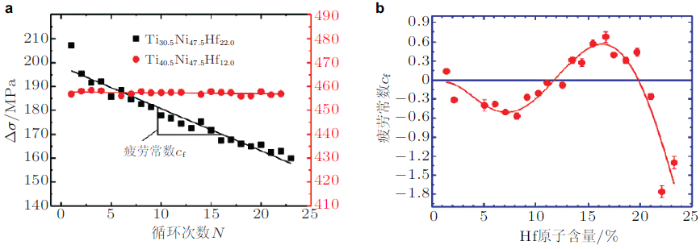## 4 磁致形状记忆合金的磁--力耦合循环变形行为

### 4.1 实验研究

4.1.1 磁--力耦合变形机理分析

(1)磁场诱发马氏体重定向：Tickle和 James (1999), Tickle 等(1999), Heczko (2005), Straka 和 Heczko (2003)以及Karaca等(2006)研究了环境温度在马氏体完成温度$$(M_{\rm f})$$以下时铁磁形状记忆合金单晶在轴向恒定压应力、横向交变磁场作用下磁场诱发马氏体重定向特性.此时, 材料的初始相为马氏体, 图43给出了该加载工况下典型的磁场--相对磁化曲线及磁场--应变曲线.以压应力为1.4 MPa的工况为例, 由图可见: 在变形的初始阶段(即磁场低于0.5 T), 由于磁场低于马氏体重定向临界磁场, 无非弹性应变出现, 并且磁化量随着磁场的增大而线性增大, 该阶段的磁化机制主要为磁化矢量的旋转; 当磁场高于0.5 T时, 马氏体重定向发生, 伴随着很大的非弹性应变出现, 同时磁化率出现跳跃并远高于低磁场(小于0.5 T)时的磁化率, 在该阶段马氏体重定向和磁化矢量旋转这两种磁化机制同时发生; 当磁场达到0.8 T时, 马氏体重定向完成, 磁化饱和出现, 并在后续磁场加载过程中, 非弹性应变和磁化量不再变化; 在卸载过程中, 当磁场达到0.25 T时, 逆向重定向发生, 非弹性应变和磁化量剧烈变化; 当磁场完全卸载后, 马氏体重定向完成, 非弹性应变及磁化量完全归零.另外, 对比图43中不同应力水平下的磁--力响应可以看出：磁致伸缩应变的大小随着轴向压应力的增大而逐渐减小, 并且轴向压应力对磁场诱发马氏体重定向有抑制作用, 即临界磁场强度随着轴向压应力的增加而逐渐升高; 在压应力较小时(0.6 MPa), 磁场诱发出的重定向应变无法完全恢复; 在压应力较大时(3 MPa), 试样在发生马氏体重定向之前已经通过磁化矢量旋转机制完成了磁化, 无法诱发马氏体重定向机制. 这说明, 铁磁形状记忆合金存在着一个"闭锁应力", 在应力低于"闭锁应力"时, 磁场能够诱发马氏体重定向; 在应力高于"闭锁应力"时, 磁场无法诱发马氏体重定向. 铁磁形状记忆合金的"闭锁应力"一般较低, 大约在3 MPa左右. Karaca等(2006)进一步提出了发生马氏体重定向的两个必要条件, 即高的磁晶各向异性能和低的孪生界面运动能, 并通过增大测试温度与居里温度之差以及减小测试温度和马氏体相变温度之差分别提高和降低磁晶各向异性能和孪生界面运动能, 进而获得了较高的应力闭锁值(5.7 MPa).(2)应力诱发马氏体重定向. Heczko (2005), Karaca 等(2006), Chen等(2014)Heidi 等(2016)研究了环境温度在马氏体完成温度$$M_{\rm f}$$以下时铁磁形状记忆合金单晶在横向恒定磁场、轴向交变压应力作用下应力诱发马氏体重定向特性.图44给出了在该加载工况下典型的应力应变曲线. 由图可见: 在无磁场作用下时, 加载诱发出的非弹性应变在应力完全卸载后不能恢复, 这是由于马氏体逆向重定向开始应力大于零; 而在磁场作用下, 马氏体重定向平台随着磁场的增大的逐渐升高, 这表明横向磁场对轴向应力诱发马氏体重定向有抑制作用; 当磁场达到8 kG时, 重定向应变在应力卸载后能够完全恢复, 材料展示出超弹性效应. 需要说明的是, 铁磁形状记忆合金在$$M_{\rm f}$$以下的超弹性机制是正向和逆向马氏体重定向, 这与NiTi形状记忆合金在奥氏体完成温度$$A_{\rm f}$$以上由正向和逆向马氏体相变所导致的超弹性有本质区别.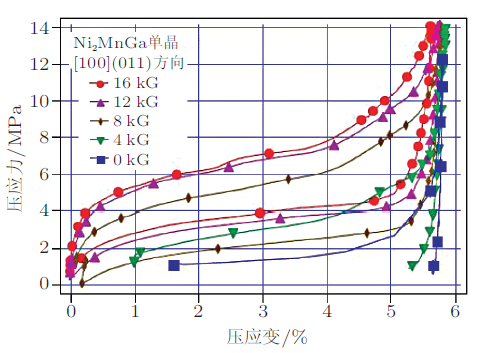(3)应力诱发马氏体相变. Chernenko 等(2005), Segu\'{\i} 等(2005), Kim 等(2006)Karaca 等(2007)研究了环境温度在奥氏体完成温度A$$_{\rm f}$$以上时铁磁形状记忆合金单晶在横向恒定磁场、轴向交变压应力作用下应力诱发马氏体相变特性.和NiTi形状记忆合金类似, 铁磁形状记忆环境在应力作用下展示出良好的超弹性特性, 如图45所示. 同时, 可以看出横向磁场对马氏体相变具有抑制作用, 即向前相变和逆相变平台均随着横向磁场的增加而升高, 并且相变特性对温度有很强的依赖性. 需要说明的是, 为了便于比较, 图43中每个温度下的应变初始值均为零, 坐标轴的刻度间隔为1%.(4)磁场诱发马氏体相变. 与马氏体重定向相比, 单纯的磁场诱发马氏体相变十分困难, 需要的临界磁场通常高于5T(Kakeshita et al. 2000, Haldar et al. 2014), 这极大地限制了铁磁形状记忆合金的应用范围.为了能使铁磁形状记忆合金在较低磁场下仍然能够诱发马氏体相变机制, Karaman 等(2006)和Karaca 等(2007)提出了一种行之有效的方法, 即应力辅助磁场诱发马氏体相变：首先施加应力, 使试样发生马氏体相变, 然后卸载至无磁场下逆相变开始应力和1.6 T磁场下逆相变开始应力之间, 如图46所示. 此时, 保持应力不变, 施加磁场, 即可在较小的磁场强度下(0.8 T)诱发马氏体相变机制, 同时可以观察到较大的相变应变, 如图44所示. 需要指出的是, 磁场诱发马氏体相变是一种很重要的机制, 这是由于在同样磁场强度下, 磁场诱发马氏体相变的输出功率远高于磁场诱发马氏体重定向, 这对铁磁形状记忆合金磁驱动器的设计提供了新的理论基础.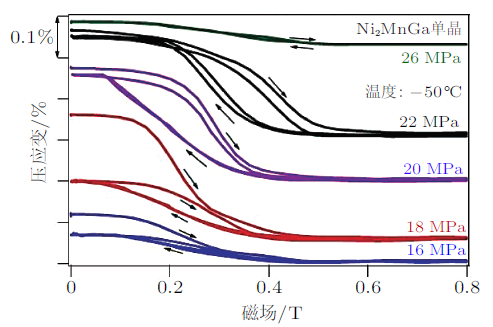4.1.2 磁--力耦合循环变形行为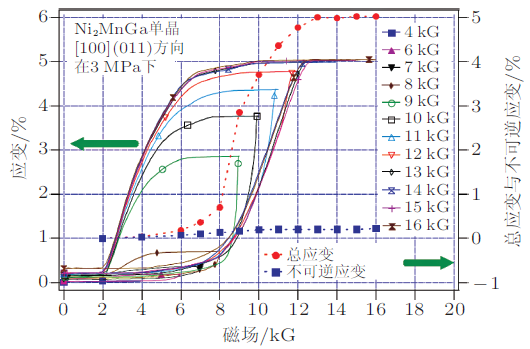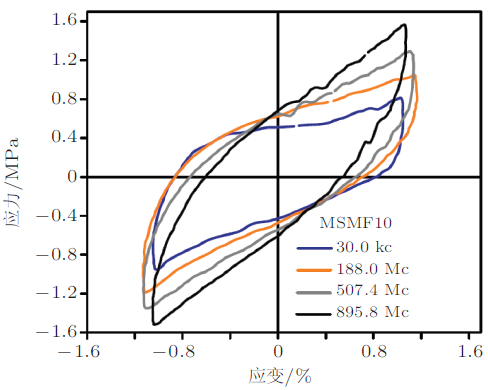### 4.2 磁--力耦合本构理论

(3)基于不可逆热力学的宏观唯象模型.该类模型通过引入内变量并结合外变量来表征材料的热力学状态, 通过建立的Helmholtz或Gibbs自由能, 采用热力学第二定律推导出各种非弹性变形机制生成的耗散, 进而定义与内变量共轭的热力学驱动力, 最后基于热力学约束和实验观察, 提出内变量演化方程, 得到描述材料本构关系的封闭微分方程组.这类模型由于概念较为简单, 便于工程应用, 目前得到了较为广泛的关注.(4)基于Eshelby夹杂理论的细观力学模型.该类模型将初始相为马氏体相的铁磁形状记忆合金中的一个变体看作基体, 另外一个变体看作夹杂, 通过引入Eshelby张量度量材料内部应力--应变场的非均匀性, 并采用Mori-Tanaka方法得到材料的宏观性能.这类模型物理概念清晰、能够反映铁磁形状记忆合金的细观非均匀性以及微结构的交互作用, 进而具有良好的发展前景.

4.2.1 宏观唯象本构理论

Mousavi 和 Arghavani (2017)考虑了三种与磁致应变相关的变形机制, 即马氏体重定向、畴壁运动和磁畴旋转, 因此, 在本构方程中引入了三个马氏体变体体积分数(即 $$\xi _{1}$$, $$\xi _{2}$$和 $$\xi _{3})$$, 第$$i$$个马氏体变体中的两种磁畴体积分数(即$$\alpha _{i}$$和$$1-\alpha _{i})$$和第$$i$$个马氏体变体中两种磁畴中磁化矢量方向$$(\pmb m_{ +i} , \pmb m_{ - i})$$作为内变量来描述材料所处的热力学状态, 系统的自由能$$g$$写成$g=g^{\rm mec} + g^{\rm mag} + g^{\rm T} +g^{\rm int} + g^{\rm con} \ \ (51)$ 其中, $$g^{\rm mec}$$, $$g^{\rm mag}$$, $$g^{\rm T}$$, $$g^{\rm int}$$和$$g^{\rm con}$$分别是力学能、磁能、温度相关内能、硬化能以及约束能.它们的具体形式为$g^{\rm mec}=-\dfrac{1}{2\rho}\pmb\sigma:\pmb S:\pmb\sigma-\dfrac{1}{\rho}\pmb\sigma:\pmb \varepsilon^{\rm r} \ \ (52)$$g^{\rm mag}=-\dfrac{\mu_0}{\rho}\pmb M\cdot\pmb H+\sum_{i=1}^3\xi_i\alpha_iK_I\left[1-(\pmb e_i\cdot\pmb m_{+i})^2\right]+ \sum_{i=1}^3\xi_i(1-\alpha_i)K_I\left[1-(\pmb e_i\cdot\pmb m_{-i})^2\right] \ \ (53)$$g^{\rm T}=C_p\left[T-T_0-T\ln\left(\dfrac{T}{T_0}\right)\right]\ \ (54)$$g^{\rm int}=\dfrac{1}{\rho n}k_1\left(\xi_1^n +\xi_2^n +\xi_3^n \right) \ \ (55)$$g^{\rm con}=\dfrac{1}{\rho } [\lambda_\xi \left(\xi_1 +\xi_2 +\xi_3-1\right)+I^{\xi_1}(\xi_1)+I^{\xi_2}(\xi_2)+I^{\xi_3}(\xi_3)+I^{\alpha_1}(\alpha_1)+I^{\alpha_2}(\alpha_2)+$$I^{\alpha_3}(\alpha_3)+\lambda_{+1}(\|\pmb m_{+1}\|-1)+\lambda_{-1}(\|\pmb m_{-1}\|-1)+\lambda_{+2}(\|\pmb m_{+2}\|-1)+$$\lambda_{-2}(\|\pmb m_{-2}\|-1) + \lambda_{+3}(\|\pmb m_{+3}\|-1)+\lambda_{-3}(\|\pmb m_{-3}\|-1) \ \ (56)$ 其中, $$\rho$$是材料密度, $$\pmb\sigma$$是应力张量, $$\pmb S$$是柔度张量, $$\pmb \varepsilon ^{r}$$是重定向应变张量, $$\mu_{0}$$是真空磁导率, $$\pmb M$$是磁化矢量, $$\pmb H$$是磁场强度; $$\pmb e_{i}$$是坐标基础矢量; $$C_{p}$$是比热容, $$T_{0}$$是平衡温度; $$K_{I}$$, $$n$$, $$k_{1}$$是材料参数; $$\lambda_{\xi }$$, $$\lambda_{ + 1}$$, $$\lambda_{ - 1}$$, $$\lambda_{ + 2}$$, $$\lambda_{ - 2}$$, $$\lambda_{ + 3}$$和 $$\lambda _{ -3}$$是拉格朗日乘子; $$I^{\xi i} ( \xi _{i})$$和$$I^{\alpha i} (\alpha_{i})$$是约束函数.

Mousavi 和 Arghavani(2017)进一步根据热力学关系推导出与每个马氏体变体对应的热力学驱动力, 即$\pi ^{\xi_i}=-\rho\dfrac{\partial g}{\partial \xi_i}=-\varepsilon^{r,\max}\sigma_{ii}+\mu_0 M^{\rm sat}\left\{\left[\alpha_i\pmb m_{ + i}+(1-\alpha_i)\pmb m_{-i}\right]\cdot\pmb H\right\}-$$\rho K_I \alpha_i\left\{1-\left(\pmb e_i\cdot\pmb m_{ + i}\right)^2\right\}- \rho K_I(1-\alpha_i)\left\{1-\left(\pmb e_i\cdot\pmb m_{-i}\right)^2\right\}-k_1\xi_i^{n-1}-\lambda_\xi-\dfrac{\partial I^{\xi_i}( \xi_i)}{\partial \xi_i} \ \ (59)$ 同时, 将马氏体重定向的驱动力定义为最大的马氏体变体驱动力和最小的马氏体变体驱动力之差, 并认为当驱动力之差达到一个定值之后, 马氏体重定向发生, 即$F=\pi ^{\xi p}-\pi ^{\xi R}=Y \ \ (60)$ 其中, $$\pi ^{\xi p}$$和 $$\pi ^{\xi R}$$分别是最大的和最小的马氏体变体热力学驱动力.

Mousavi 和 Arghavani (2017)对提出的本构模型进行了数值实现, 并通过将模拟结果和实验结果的对比验证了模型的正确性. 图49图50分别给出了Mousavi 和Arghavani模型(Mousavi & Arghavani 2017)、Chen模型 (Chen et al. 2014)以及LaMaster模型(LaMaster et al. 2014)在应力保持下$$(-1.4$$ MPa)磁场诱发重定向导致的磁致应变实验结果(Heczko 2005)和应力诱发重定向实验结果 (Chen et al. 2014)与对应的模拟结果的比较情况. 由图可以看出, 三个模型都能够较好地捕捉到实验现象, 但是, Mousavi 和 Arghavani模型(Mousavi & Arghavani 2017)与实验数据吻合得更好.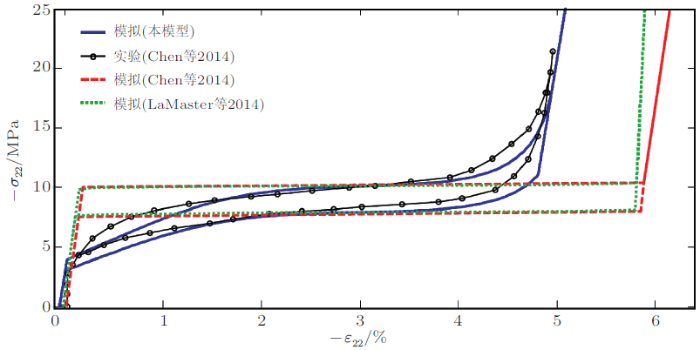4.2.2 基于Eshelby夹杂理论的细观力学本构理论

Zhu 和 Dui (2007)将第一个马氏体变体视为基体, 第二个马氏体视为夹杂, 并将宏观整体应变$$\bar{\pmb\varepsilon}$$分解为弹性应变$$\bar{\pmb\varepsilon }^{\rm e}$$和非弹性应变$$\bar{\pmb\varepsilon }^{\rm in}$$, 即 $\bar{\pmb\varepsilon } = \bar{\pmb\varepsilon }^{\rm e} +\bar{\pmb\varepsilon }^{\rm in} \ \ (61)$其中, $$\bar{\pmb\varepsilon }^{\rm e}$$和$$\bar{\pmb\varepsilon }^{\rm in}$$分别可以表示为 $\bar{\pmb\varepsilon }^{\rm e} = \overline{\pmb M}\bar {\pmb \sigma } \ \ {\rm (62a)}$ $\bar{\pmb\varepsilon }^{\rm in} = \xi \left( {1 - \xi} \right)\left\{ {\pmb L_0 + \left( {\pmb L_1 - \pmb L_0 }\right)\left[ {\pmb S - \xi \left( {\pmb S - \pmb I} \right)}\right]} \right\}^{ - 1}\left( {\pmb L_0 - \pmb L_1 }\right)\left( {\pmb S - \pmb I} \right)\left( {\pmb \varepsilon^{\rm r} - \pmb \varepsilon ^{\rm ph}} \right)+$ $\pmb \varepsilon^{\rm ph} + \xi \pmb \varepsilon ^{\rm r} \ \ {\rm (62b)}$其中, $$\xi$$是第二个马氏体变体的体积分数, $$\pmb L_0$$和$$\pmb L_1$$分别是基体和夹杂的弹性柔度张量, $$\pmb S$$是Eshelby张量, $$\pmb I$$是四阶单位张量, $$\pmb \varepsilon ^{\rm r}$$和$$\pmb \varepsilon^{\rm ph}$$分别是重定向和相变应变张量, $$\bar {\pmb\sigma }$$是宏观整体应力. $$\overline{\pmb M}$$是宏观整体有效弹性柔度张量 $\overline{\pmb M} = \left\{ {\pmb I + \xi \left\{ {\pmb L_0 + \left( {\pmb L_1 - \pmb L_0 } \right)\left[{\pmb S - \xi \left( {\pmb S - \pmb I} \right)} \right]}\right\}^{ - 1}\left( {\pmb L_0 - \pmb L_1 } \right)} \right\}\pmb M_0 \ \ (63)$Zhu 和 Dui (2007)将两个马氏体变体的Gibbs自由能之差分成三项, 即 $\Delta G = \Delta G_{\rm me} + \Delta G_{\rm ze} + \Delta G_{\rm an} \ \ (64)$其中, $$\Delta G_{\rm me}$$, $$\Delta G_{\rm ze}$$和$$\Delta G_{\rm an}$$分别是机械能、Zeeman能和磁晶各向异性能, 即 $\Delta G_{\rm me} = - \dfrac{1}{2}\xi \left( {1 - \xi }\right)\pmb L_0 \left( {\pmb S - \pmb I} \right)\pmb \varepsilon^{\rm r}\pmb \varepsilon ^{\rm r} \ \ {\rm (65a)}$ $\Delta G_{\rm ze} = \left( {1 - \xi } \right)\mu _0 M^{\rm sat}H\left\{ \left[{\alpha \cos \beta _3 - \left( {1 - \alpha } \right)\cos \beta _1} \right]\cos \theta +\right.$ $\left. \left[ {\alpha \sin \beta _3 - \left( {1 -\alpha } \right)\sin \beta _1 } \right]\sin \theta \right\} + \xi \mu _0 M^{\rm sat}H\left\{ \left[ { - \alpha \sin \beta _4 +\left( {1 - \alpha } \right)\sin \beta _2 } \right]\cos \theta+\right.$ $\left.\left[ {\alpha \cos \beta _4 - \left( {1 - \alpha } \right)\cos\beta _2 } \right]\sin \theta \right\}\ \ {\rm (65b)}$ $\Delta G_{\rm an} = \left( {1 - \xi } \right)\left[{\alpha K_u \sin ^2\beta _3 + \left( {1 - \alpha } \right)K_u \sin^2\beta _1 } \right] +$ $\xi \left[ {\alpha K_u \sin ^2\beta _4 +\left( {1 - \alpha } \right)K_u \sin ^2\beta _2 } \right]\ \ {\rm (65c)}$ 式中, $$\beta_1$$和$$\beta_2$$为第一个马氏体变体中两种磁畴磁化矢量与磁化易轴的夹角, $$\beta_3$$和$$\beta_4$$为第二个马氏体变体中两种磁畴磁化矢量与磁化易轴的夹角, $$K_u$$为磁晶各向异性能, $$\alpha$$和$$1-\alpha$$为马氏体变体中两种磁畴的体积分数.

Zhu 和 Dui (2007)将两个马氏体变体的Gibbs自由能之差对体积分数$$\xi$$的导数作为马氏体重定向的驱动力, 即 $f_{\rm drv} = - \dfrac{\partial \Delta G}{\partial \xi }= - \dfrac{\partial \Delta G_{\rm me} }{\partial \xi } -\dfrac{\partial \Delta G_{\rm ze} }{\partial \xi } -\dfrac{\partial \Delta G_{\rm an} }{\partial \xi } \ \ (66)$进一步考虑了两种马氏体重定向的能垒, 即界面能和界面摩擦耗散能 $\Delta G_{\rm s} = {2\xi \gamma _{\rm s} } /t \ \ {\rm (67a)}$ $\Delta G_{\rm d} = D\left( {e^{b\xi } - 1} \right)\ \ {\rm (67b)}$其中, $$\gamma _{\rm s}$$是马氏体--马氏体界面能密度, $$t$$是界面厚度, $$D$$和$$b$$是材料参数. 同时, 将两种能垒之和对体积分数$$\xi$$的导数作为马氏体重定向的阻力 $f_{\rm res} = \dfrac{\partial \Delta G_{\rm s} }{\partial \xi }+ \dfrac{\partial \Delta G_{\rm d} }{\partial \xi } \ \ (68)$同时, 提出如下马氏体重定向演化准则 $f_{\rm drv} = f_{\rm res} \ \ (69)$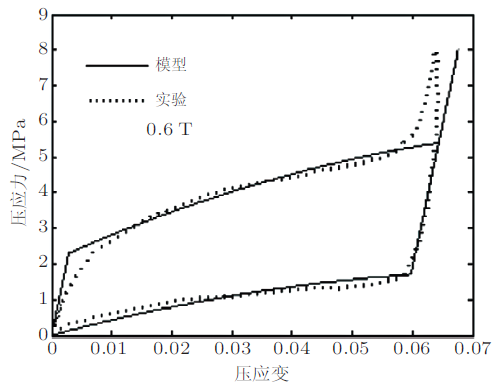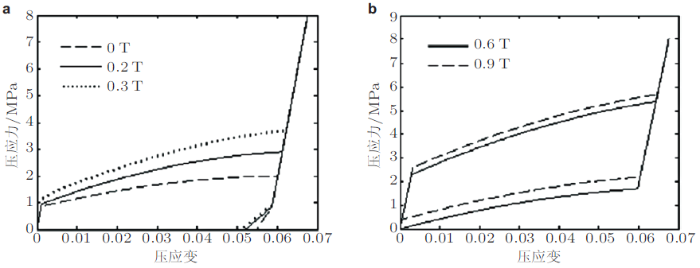## 5 磁致形状记忆合金的磁--力耦合疲劳失效行为

(1)磁场诱发疲劳失效. Chmielus等(2008)通过对NiMnGa单晶施加循环旋转磁场(磁场强度保持恒定, 方向改变)观察了磁致应变在循环变形过程中的演化, 如图53所示.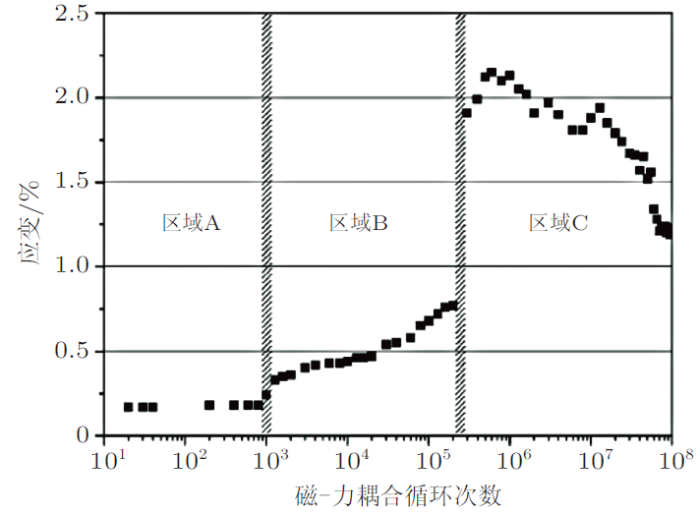Guldbakke 等(2010)也研究了NiMnGaFe单晶在相同加载模式下的疲劳特性, 结果表明, NiMnGaFe单晶的磁致应变演化特性和NiMnGa单晶非常类似, 也分为上述三个阶段, 但其抗疲劳特性较差, 试样在经历2 $$\times$$ 10$$^{6}$$次循环磁场载荷后出现断裂.Lawrence 等(2016)则观察了NiMnGa单晶在磁场诱发疲劳后的试样微观形貌, 发现三种典型的裂纹形式同时存在, 即: I型裂纹沿$$<100>$$方向扩展, II型裂纹沿$$<100>$$方向扩展, III型裂纹沿小角度晶界扩展, 如图54所示(图中所示的1, 2和3分别代表I型、II型和III型裂纹).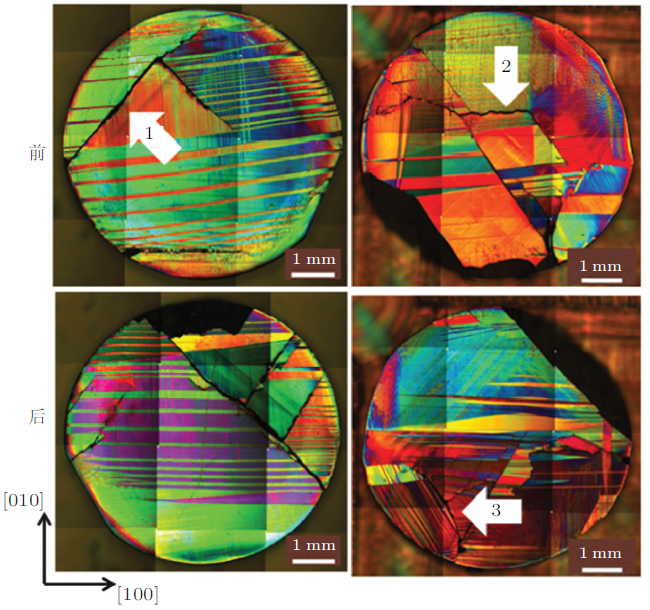(2)机械载荷诱发疲劳失效. Aaltio等(2010)研究了机械循环载荷作用下NiMnGa单晶的疲劳特性, 所得的典型循环应力--应变曲线如图48所示. 由图可见, 与普通NiTi形状记忆合金类似, NiMnGa单晶在循环变形中也会出现明显的功能性劣化, 即重定向开始应力和重定向模量均随着循环次数的增大而升高. Aaltio等(2010)指出, 上述的功能性劣化现象来源于两个方面: 一是在机械循环载荷作用下, 马氏体孪晶结构逐渐从大孪晶转变为细孪晶, 导致新孪晶形成后自由运动空间变小, 从而增大了新孪晶的运动阻力, 如图55所示; 二是在循环变形过程中, 晶体缺陷(如位错、空洞)逐渐累积, 这些缺陷的存在增大了材料内部的应力场梯度, 从而阻碍了马氏体孪生界面运动, 从而造成重定向模量逐渐升高. 同时, Aaltio 等(2010)的研究还表明: 材料的疲劳寿命随着应变幅值的增大而显著降低; 由于马氏体重定向机制是二级相变, 相变前后不涉及到潜热的释放, 因此材料对加载频率的依赖性很低; 虽然在循环变形过程中应力--应变曲线发生明显改变, 然而马氏体相变温度和居里温度保持不变; 材料中加工时预先存在的一些裂纹易于成为继发的裂纹源, 然而, 裂纹的扩展方向并不平行于预先存在的裂纹方向, 而是沿着马氏体孪晶的(101)晶面扩展, 因此, 在宏观上可以观察到裂纹与外加应力方向呈45$$^\circ$$角, 如图56所示.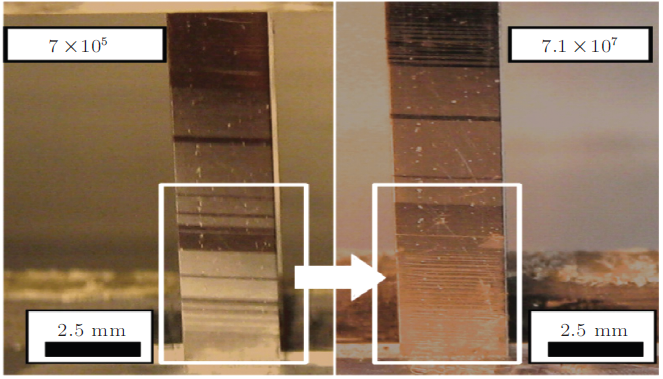## 6 总结与展望

(1)热致NiTi形状记忆合金.目前对NiTi形状记忆合金热--力耦合循环变形行为和疲劳失效的研究最为广泛, 已经在循环载荷作用下的超弹性和形状记忆退化效应(功能疲劳)和相关疲劳失效行为研究方面取得了较为丰硕的成果, 积累了很多宝贵的实验数据, 并已建立了一系列的热--力耦合本构模型和疲劳失效模型. 然而, 由于NiTi形状记忆合金循环变形行为的强烈热--力耦合特性和相变过程的局部失稳特性等, 目前对热--力耦合循环变形的微观机理以及损伤演化规律还未得到清楚的认识, 因而, 建立的理论模型预测精度还不够, 还有较大的改进空间.

(2)热致高温NiTiX形状记忆合金. 与NiTi形状记忆合金相比, 高温NiTiX形状记忆合金的热--力耦合循环变形和疲劳失效行为的研究要少很多, 目前已有的研究主要集中在恒定应力的温度循环方面, 并且局限于宏观循环变形实验和本构模型研究方面, 对于变形的微观机理和热--力耦合疲劳失效方面涉及甚少, 还需进行系统而深入的研究. 另外, 即使是在宏观唯象的本构模型研究方面, 已有的模型大都是基于NiTi形状记忆合金的本构模型拓展而来, 专门针对NiTiX高温形状记忆合金热--力耦合循环变形行为的本构模型研究较少.

(3)磁致形状记忆合金.

(1)开展热致和磁致形状记忆合金的热--力和磁--热--力耦合循环变形和疲劳失效行为的宏微观实验, 积累丰富的第一手实验数据.

(2)结合宏微观实验观察, 利用分子动力学和相场分析以及有限元分析等多尺度数值模拟方法, 探究热致和磁致形状记忆合金循环变形和失效行为的微观机理, 揭示形状记忆合金复杂的微观结构与宏观力学行为的关系, 优化材料设计.

(3)基于揭示的微观机理, 建立热致和磁致形状记忆合金的热--力和磁--热--力耦合循环本构模型和疲劳失效模型.

(4)基于建立的理论模型, 开展热致和磁致形状记忆合金器件的多场耦合分析, 对其服役寿命和可靠性进行评价.

The authors have declared that no competing interests exist.

## 参考文献 原文顺序 文献年度倒序 文中引用次数倒序 被引期刊影响因子

  康国政, 于超, 阚前华. 2015. NiTi 形状记忆合金热--力耦合循环变形行为宏微观实验和理论研究进展. 固体力学学报, 36: 461-480 (Kang G Z, Yu C, Kan Q H.2015. Progress in thermo-mechanical cyclic deformation of NiTi shape memory alloy: Macroscopic/Microscopic experiments and theory. Chinese Jounral of Solid Mechanics,36: 461-480).  康国政. 2011. 超弹性镍钛形状记忆合金循环变形行为的研究进展. 西南交通大学学报, 46: 355-364 (Kang G Z.2011. Research progress in cyclic deformation of super-elastic NiTi shape memory alloy. Journal of Southwest Jiaotong University,46: 355-364).  宋迪. 2015. 超弹性 NiTi 形状记忆合金微管的疲劳行为及损伤演化模型研究. [博士论文]. 成都: 西南交通大学 (Song D.2015. Study on the fatigue behavior and damage evolution model of super-elastic NiTi shape memory alloy microtubes. [PhD Thesis]. Chengdu: Southwest Jiaotong University).  王习术. 2010. 材料力学行为试验与分析 (第2版). 北京: 清华大学出版社, 77-91 (Wang X S.2010. Test and Analysis on Mechanical Behavior of Materials (Second Edition). Beijing: Tsinghua University Press, 77-91).  赵连城, 蔡伟, 郑玉峰. 2002.合金的形状记忆合金效应与超弹性.第一版. 北京:国防工业出版社 (Zhao L C, Cai W, Zheng Y F.2002. Shape Memory Effect and Superelasticity of Alloys. (First Edition). Beijing: National Defence Industry Press) .  左舜贵, 金学军, 金明江. 2014. 高温形状记忆合金的研究进展. 机械工程材料, 38: 1-5 (Zuo S G, Jin X J, Jin M J.2014. Research progress in high temperature shape memory alloys. Materials for Mechanical Engineering,38: 1-5).  周廷, 阚前华, 康国政, 邱博. 2017. 超弹性镍钛形状记忆合金单轴相变棘轮行为的宏观唯象本构模型. 力学学报, 49: 588-596 (Zhou T, Kan Q H, Kang G Z, Qiu B.2017. A macroscopic phenomenological constitutive model for the uniaxial transformation ratcheting of super-elastic NiTi shape memory alloy. Chinese Journal of Theoretical and Applied Mechanics,49: 588-596).  Aaltio I, Soroka A, Ge Y, Söderberg O, Hannula S P.2010. High-cycle fatigue of 10M Ni-Mn-Ga magnetic shape memory alloy in reversed mechanical loading. Smart Mater. Struct., 19: 075014.  Ahadi A, Sun Q.2013. Stress hysteresis and temperature dependence of phase transition stress in nanostructured NiTi---Effects of grain size. Appl. Phys. Lett., 103: 021902.  Ahadi A, Sun Q.2014. Effects of grain size on the rate-dependent thermomechanical responses of nanostructured superelastic NiTi. Acta Mater., 76: 186-197.  Allen S M, Cahn J W.1979. A microscopic theory for antiphase boundary motion and its application to antiphase domain coarsening. Acta Metall., 27: 1085-1095.  Auricchio F, Bessoud A-L, Reali A, Stefanelli U.2015. A phenomenological model for the magneto-mechanical response of single-crystal magnetic shape memory alloys. Eur. J. Mech. A-Solid, 52: 1-11.  Auricchio F, Fugazza D, Desroches R.2008. Rate-dependent thermomechanical modelling of superelastic shape-memory alloys for seismic applications. J. Intell. Mater. Syst. Struct., 19: 47-61.  Benafan O, Noebe R D, Ii S A P, Brown D W, Vogel S, Vaidyanathan R.2014. Thermomechanical cycling of a NiTi shape memory alloy-macroscopic response and microstructural evolution. Int. J. Plast., 56: 99-118.  Bigelow G S, Padula Ii S A, Garg A, Noebe R D.2007. Correlation between mechanical behavior and actuator-type performance of Ni-Ti-Pd high-temperature shape memory alloys. International Symposium on: Smart Structures & Materials & Nondestructure Evaluation & Health Monitorying, 6526: 6526B-6526B-12.  Bigelow G S, Padula S A, Garg A, Gaydosh D, Noebe R D.2010. Characterization of ternary nitipd high-temperature shape-memory alloys under load-biased thermal cycling. Metall. Mater. Trans. A, 41: 3065-3079.  Bo Z, Lagoudas D C.1999. Thermomechanical modeling of polycrystalline SMAs under cyclic loading, Part III: Evolution of plastic strains and two-way shape memory effect. Int. J. Eng. Sci., 37: 1175-1203.  Cahn J W, Allen S M.1977. Microscopic theory for domain wall motion and its experimental verification in fe-al alloy domain growth kinetics. J. Phy. Colloques, 38: C7-51-C7-54.  Cahn J W, Hilliard J E.1958. Free energy of a nonuniform system. I. Interfacial free energy. J. Chem. Phys., 28: 258-267.  Cahn J W, Hilliard J E.1959. Free energy of a nonuniform system. iii. nucleation in a two---component incompressible fluid. J. Chem. Phys., 31: 688-699.  Charkaluk E, Bignonnet A, Constantinescu A, Dang Van K.2002. Fatigue design of structures under thermomechanical loadings. Fatigue Fract. Eng. Mater. Struct., 25: 1199-1206.  Charkaluk E, Constantinescu A.2000. An energetic approach in thermomechanical fatigue for silicon molybdenum cast iron. Mater. High Temp., 17: 373-380.  Chemisky Y, Chatzigeorgiou G, Kumar P, Lagoudas D C.2014. A constitutive model for cyclic actuation of high-temperature shape memory alloys. Mech. Mater., 68: 120-136.  Chen X, Moumni Z, He Y, Zhang W.2014. A three-dimensional model of magneto-mechanical behaviors of martensite reorientation in ferromagnetic shape memory alloys. J. Mech. Phys. Solids, 64: 249-286.  Chernenko V A, Pons J, Cesari E, Ishikawa K.2005. Stress-temperature phase diagram of a ferromagnetic Ni-Mn-Ga shape memory alloy. Acta Mater., 53: 5071-5077.  Chmielus M, Chernenko V A, Knowlton W B, Kostorz G, Müllner P.2008. Training, constraints, and high-cycle magneto-mechanical properties of Ni-Mn-Ga magnetic shape-memory alloys. Eur. Phys. J. Spec. Top., 158: 79-85.  Christ D, Reese S.2009. A finite element model for shape memory alloys considering thermomechanical couplings at large strains. Int. J. Solids. Struct., 46: 3694-3709.  Chulist R, Skrotzki W, Oertel C G, Böhm A, Pötschke M.2010. Change in microstructure during training of a Ni50Mn29Ga21 bicrystal. Scripta Mater., 63: 548-551.  Cisse C, Zaki W, Ben Zineb T.2016. A review of constitutive models and modeling techniques for shape memory alloys. Int. J. Plast., 76: 244-284.  Creton N, Hirsinger L.2005. Rearrangement surfaces under magnetic field and/or stress in Ni-Mn-Ga. J. Magn. Magn. Mater., 290-291: 832-835.  Delville R, Malard B, Pilch J, Sittner P, Schryvers D.2010. Microstructure changes during non-conventional heat treatment of thin Ni-Ti wires by pulsed electric current studied by transmission electron microscopy. Acta Mater., 58: 4503-4515.  Delville R, Malard B, Pilch J, Sittner P, Schryvers D.2011. Transmission electron microscopy investigation of dislocation slip during superelastic cycling of Ni-Ti wires. Int. J. Plast., 27: 282-297.  DeSimone A, James R D.2002. A constrained theory of magnetoelasticity. J. Mech. Phys. Solids, 50: 283-320.  Dhote R P, Gomez H, Melnik R N V, Zu J.2015. 3D coupled thermo-mechanical phase-field modeling of shape memory alloy dynamics via isogeometric analysis. Comput. Struct., 154: 48-58.  Di Cocco V, Iacoviello F, Maletta C, Natali S.2014. Cyclic microstructural transitions and fracture micromechanisms in a near equiatomic NiTi alloy. Int. J. Fatigue, 58: 136-143.  Duerig T, Pelton A, Stöckel D.1999. An overview of nitinol medical applications. Mater. Sci. Eng. A, 273-275: 149-160.  Dunand-Ch\^{a}tellet C, Moumni Z.2012. Experimental analysis of the fatigue of shape memory alloys through power-law statistics. Int. J. Fatigue, 36: 163-170.  Eggeler G, Hornbogen E, Yawny A, Heckmann A, Wagner M.2004. Structural and functional fatigue of NiTi shape memory alloys. Mater. Sci. Eng. A, 378: 24-33.  Falk F.1983a. Ginzburg-Landau theory of static domain walls in shape-memory alloys. Z. Phys. B, 51: 177-185.  Falk F.1983b. One-dimensional model of shape memory alloys. Arch. Mech, 35: 63-84.  Fan D, Chen L Q.1997. Computer simulation of grain growth using a continuum field model. Acta Mater., 45: 611-622.  Feng P, Sun Q P.2006. Experimental investigation on macroscopic domain formation and evolution in polycrystalline NiTi microtubing under mechanical force. J. Mech. Phys. Solids, 54: 1568-1603.  Franciosi P.1985. The concepts of latent hardening and strain hardening in metallic single crystals. Acta Metall., 33: 1601-1612.  Frotscher M, Neuking K, Böckmann R, Wolff KD, Eggeler G.2008. In situ scanning electron microscopic study of structural fatigue of struts, the characteristic elementary building units of medical stents. Mater. Sci. Eng. A, 481-482: 160-165.  Gauthier J Y, Lexcellent C, Hubert A, Abadie J, Chaillet N.2006. Modeling rearrangement process of martensite platelets in a magnetic shape memory alloy Ni$$_{2}$$MnGa single crystal under magnetic field and (or) stress action. J. Intell. Mater. Syst. Struct., 18: 289-299.  Grabe C, Bruhns O T.2008. On the viscous and strain rate dependent behavior of polycrystalline NiTi. Int. J. Solids. Struct., 45: 1876-1895.  Grandi D, Maraldi M, Molari L.2012. A macroscale phase-field model for shape memory alloys with non-isothermal effects: Influence of strain rate and environmental conditions on the mechanical response. Acta Mater., 60: 179-191.  Guldbakke J M, Chmielus M, Rolfs K, Schneider R, Müllner P, Raatz A.2010. Magnetic, mechanical and fatigue properties of a Ni$$_{45.4}$$Mn$$_{29.1}$$Ga$$_{21.6}$$Fe$$_{3.9}$$ single crystal. Scripta Mater., 62: 875-878.  Guo X H, Shi S-Q, Ma X Q.2005. Elastoplastic phase field model for microstructure evolution. Appl. Phys. Lett., 87: 221910.  Haldar K, Lagoudas D C, Karaman I.2014. Magnetic field-induced martensitic phase transformation in magnetic shape memory alloys: Modeling and experiments. J. Mech. Phys. Solids, 69: 33-66.  Hamilton R F, Sehitoglu H, Chumlyakov Y, Maier H J.2004. Stress dependence of the hysteresis in single crystal NiTi alloys. Acta Mater., 52: 3383-3402.  Hartl D J, Chatzigeorgiou G, Lagoudas D C.2010. Three-dimensional modeling and numerical analysis of rate-dependent irrecoverable deformation in shape memory alloys. Int. J. Plast., 26: 1485-1507.  He Y J, Chen X, Moumni Z.2011. Two-dimensional analysis to improve the output stress in ferromagnetic shape memory alloys. J. Appl. Phys., 110: 063905.  He Y J, Sun Q P.2010a. Rate-dependent domain spacing in a stretched NiTi strip. Int. J. Solids. Struct., 47: 2775-2783.  He Y J, Sun Q P.2010b. Frequency-dependent temperature evolution in NiTi shape memory alloy under cyclic loading. Smart Mater. Struct., 19: 115014.  Hebda D A, White S R.1998. Effect of training conditions and extended thermal cycling on nitinol two-way shape memory behavior. Smart Mater. Struct., 4: 298.  Heczko O.2005. Magnetic shape memory effect and magnetization reversal. J. Magn. Magn. Mater., 290-291: 787-794.  Heidi P F, Constantin C, Eberle J L, Jason L D.2016. Experimental characterization and modeling of a three-variant magnetic shape memory alloy. Smart Mater. Struct., 25: 104004.  Hirsinger L, Lexcellent C.2003. Modelling detwinning of martensite platelets under magnetic and (or) stress actions on Ni-Mn-Ga alloys. J. Magn. Magn. Mater., 254-255: 275-277.  James R D, Wuttig M.1998. Magnetostriction of martensite. Philos. Mag. A, 77: 1273-1299.  Javanbakht M, Levitas V I.2015. Interaction between phase transformations and dislocations at the nanoscale. Part 2: Phase field simulation examples. J. Mech. Phys. Solids, 82: 164-185.  Jeong S, Inoue K, Inoue S, Koterazawa K, Taya M, Inoue K.2003. Effect of magnetic field on martensite transformation in a polycrystalline Ni$$_{2}$$MnGa. Mater. Sci. Eng. A, 359: 253-260.  Kakeshita T, Takeuchi T, Fukuda T, Tsujiguchi M, Saburi T, Oshima R, Muto S.2000. Giant magnetostriction in an ordered Fe$$_{3}$$Pt single crystal exhibiting a martensitic transformation. Appl. Phys. Lett., 77: 1502-1504.  Kan Q H, Kang G Z, Yan W Y, Dong Y W, Yu C.2011. An energy-based fatigue failure model for super-elastic NiTi alloys under pure mechanical cyclic loading. SPIE Conference Series, 8409.  Kan Q H, Yu C, Kang G Z, Li J, Yan W Y.2016. Experimental observations on rate-dependent cyclic deformation of super-elastic NiTi shape memory alloy. Mech. Mater., 97: 48-58.  Kang G Z, Song D.2015. Review on structural fatigue of NiTi shape memory alloys: Pure mechanical and thermo-mechanical ones. Theor. Appl. Mech. Lett., 5: 245-254.  Kang G Z.2013. Advances in transformation ratcheting and ratcheting-fatigue interaction of NiTi shape memory alloy. Acta. Mech. Solida Sin, 26: 221-236.  Kang G Z, Kan Q H, Qian L, Liu Y.2009. Ratchetting deformation of super-elastic and shape-memory NiTi alloys. Mech. Mater., 41: 139-153.  Karaca H E, Acar E, Ded G S, Basaran B, Tobe H, Noebe R D, Bigelow G, Chumlyakov Y I.2013. Shape memory behavior of high strength NiTiHfPd polycrystalline alloys. Acta Mater., 61: 5036-5049.  Karaca H E, Karaman I, Basaran B, Chumlyakov Y I, Maier H J.2006. Magnetic field and stress induced martensite reorientation in NiMnGa ferromagnetic shape memory alloy single crystals. Acta Mater., 54: 233-245.  Karaca H E, Karaman I, Basaran B, Lagoudas D C, Chumlyakov Y I, Maier H J.2007. On the stress-assisted magnetic-field-induced phase transformation in Ni$$_{2}$$MnGa ferromagnetic shape memory alloys. Acta Mater., 55: 4253-4269.  Karaman I, Karaca H E, Basaran B, Lagoudas D C, Chumlyakov Y I, Maier H J.2006. Stress-assisted reversible magnetic field-induced phase transformation in Ni2MnGa magnetic shape memory alloys. Scripta Mater., 55: 403-406.  Kastner O.2012. First Principles Modelling of Shape Memory Alloys. Berlin Heidelberg: Springer.  Ke C B, Cao S, Zhang X P.2015. Phase field modeling of Ni-concentration distribution behavior around Ni$$_{4}$$Ti$$_{3}$$ precipitates in NiTi alloys. Comp. Mater. Sci., 105: 55-65.  Kiefer B, Lagoudas D C.2005. Magnetic field-induced martensitic variant reorientation in magnetic shape memory alloys. Philos. Mag., 85: 4289-4329.  Kim J H, Inaba F, Fukuda T, Kakeshita T.2006. Effect of magnetic field on martensitic transformation temperature in Ni-Mn-Ga ferromagnetic shape memory alloys. Acta Mater., 54: 493-499.  Ko W S, Maisel S B, Grabowski B, Jeon J B, Neugebauer J.2017. Atomic scale processes of phase transformations in nanocrystalline NiTi shape-memory alloys. Acta Mater., 123: 90-101.  Kockar B, Atli K C, Ma J, Haouaoui M, Karaman I, Nagasako M, Kainuma R.2010. Role of severe plastic deformation on the cyclic reversibility of a Ti$$_{50.3}$$Ni$$_{33.7}$$Pd$$_{16}$$ high temperature shape memory alloy. Acta Mater., 58: 6411-6420.  Kockar B, Karaman I, Kim J I, Chumlyakov Y.2006. A method to enhance cyclic reversibility of NiTiHf high temperature shape memory alloys. Scripta Mater., 54: 2203-2208.  König D, Zarnetta R, Savan A, Brunken H, Ludwig A.2011. Phase transformation, structural and functional fatigue properties of Ti-Ni-Hf shape memory thin films. Acta Mater., 59: 3267-3275.  Kumar P K, Lagoudas D C.2010. Experimental and microstructural characterization of simultaneous creep, plasticity and phase transformation in high-temperature shape memory alloy. Acta Mater., 58: 1618-1628.  Kundin J, Pogorelov E, Emmerich H.2015. Numerical investigation of the interaction between the martensitic transformation front and the plastic strain in austenite. J. Mech. Phys. Solids, 76: 65-83.  Kundin J, Raabe D, Emmerich H.2011. A phase-field model for incoherent martensitic transformations including plastic accommodation processes in the austenite. J. Mech. Phys. Solids, 59: 2082-2102.  Lagoudas D C.2008. Shape Memory Alloys: Modeling and Engineering Applications. Springer.  Lagoudas D C, Chatzigeorgiou G, Kumar P K.2009a. Modeling and experimental study of simultaneous creep and transformation in polycrystalline high-temperature shape memory Alloys. J. Intell. Mater. Syst. Struct., 20: 2257-2267.  Lagoudas D C, Entchev P B.2004. Modeling of transformation-induced plasticity and its effect on the behavior of porous shape memory alloys. Part I: constitutive model for fully dense SMAs. Mech. Mater., 36: 865-892.  Lagoudas D C, Miller D A, Rong L, Kumar P K.2009b. Thermomechanical fatigue of shape memory alloys. Smart Mater. Struct., 18: 085021.  LaMaster D H, Feigenbaum H P, Ciocanel C, Nelson I D.2014. A full 3D thermodynamic-based model for magnetic shape memory alloys. J. Intell. Mater. Syst. Struct., 26: 663-679.  Lawrence T, Lindquist P, Ullakko K, Müllner P.2016. Fatigue life and fracture mechanics of unconstrained Ni-Mn-Ga single crystals in a rotating magnetic field. Mater. Sci. Eng. A, 654: 221-227.  Lemaitre J, Chaboche J L.1994. Mechanics of solid materials. Cambridge University Press..  Levitas V I, Javanbakht M.2012. Advanced phase-field approach to dislocation evolution. Phys. Rev. B, 86: 140101(R).  Levitas V I, Javanbakht M.2013. Phase field approach to interaction of phase transformation and dislocation evolution. Appl. Phys. Lett., 102: 251904.  Levitas V I, Javanbakht M.2015. Interaction between phase transformations and dislocations at the nanoscale. Part 1. General phase field approach. J. Mech. Phys. Solids, 82: 287-319.  Levitas V I, Preston D L, Lee D W.2003. Three-dimensional Landau theory for multivariant stress-induced martensitic phase transformations. III. Alternative potentials, critical nuclei, kink solutions, and dislocation theory. Phys. Rev. B, 68.  Lim T J, Mcdowell D L.1999. Mechanical behavior of an Ni-Ti shape memory alloy under axial-torsional proportional and nonproportional loading. J. Eng. Mater. Technol., 121: 9-18.  Liu Y, McCormick P G.1990. Factors influencing the development of two-way shape memory in NiTi. Acta Mater., 38: 1321-1326.  Liu Y, Xie Z, Van Humbeeck J, Delaey L.1998. Asymmetry of stress-strain curves under tension and compression for NiTi shape memory alloys. Acta Mater., 46: 4325-4338.  Ma J, Karaman I, Noebe R D.2010. High temperature shape memory alloys. Int. Mater. Rev., 55: 257-315.  Mahtabi M J, Shamsaei N, Mitchell M R.2015. Fatigue of nitinol: The state-of-the-art and ongoing challenges. J. Mech. Behav. Biomed., 50: 228-254.  Maletta C, Sgambitterra E, Furgiuele F, Casati R, Tuissi A.2012. Fatigue of pseudoelastic NiTi within the stress-induced transformation regime: A modified Coffin-Manson approach. Smart Mater. Struct., 21: 112001.  Maletta C, Sgambitterra E, Furgiuele F, Casati R, Tuissi A.2014. Fatigue properties of a pseudoelastic NiTi alloy: Strain ratcheting and hysteresis under cyclic tensile loading. Int. J. Fatigue, 66: 78-85.  Malik A, Amberg G, Borgenstam A, {\AA}gren J.2013. Phase-field modelling of martensitic transformation: the effects of grain and twin boundaries. Modell. Simul. Mater. Sci. Eng., 21: 085003.  Malik A, Yeddu H K, Amberg G, Borgenstam A, {\AA}gren J.2012. Three dimensional elasto-plastic phase field simulation of martensitic transformation in polycrystal. Mater. Sci. Eng. A, 556: 221-232.  Marioni M A, O'Handley R C, Allen S M.2002. Analytical model for field-induced strain in ferromagnetic shape-memory alloy polycrystals. J. Appl. Phys., 91: 7807-7809.  Mccluskey P J, Zhao C, Kfir O, Vlassak J J.2011. Precipitation and thermal fatigue in Ni-Ti-Zr shape memory alloy thin films by combinatorial nanocalorimetry. Acta Mater., 59: 5116-5124.  Mecking H, Kocks U F.1981. Kinetics of flow and strain-hardening. Acta Metall., 29: 1865-1875.  Meng X L, Cai W, Zheng Y F, Tong Y X, Zhao L C, Zhou L M.2002. Stress-induced martensitic transformation behavior of a Ti-Ni-Hf high temperature shape memory alloy. Mater. Lett., 55: 111-115.  Meng X L, Zheng Y F, Wang Z, Zhao L C.2000. Shape memory properties of the Ti$$_{36}$$Ni$$_{49}$$Hf$$_{15}$$ high temperature shape memory alloy. Mater. Lett., 45: 128-132.  Miller D A, Lagoudas D C.2000. Thermomechanical characterization of NiTiCu and NiTi SMA actuators: Influence of plastic strains. Smart Mater. Struct., 9: 640.  Mirzaeifar R, Desroches R, Yavari A.2011. Analysis of the rate-dependent coupled thermo-mechanical response of shape memory alloy bars and wires in tension. Continuum Mech. Thermodyn., 23: 363-385.  Mirzaeifar R, Gall K, Zhu T, Yavari A, DesRoches R.2014. Structural transformations in NiTi shape memory alloy nanowires. J. Appl. Phys., 115: 194307.  Miyazaki S, Imai T, Igo Y, Otsuka K.1986. Effect of cyclic deformation on the pseudoelasticity characteristics of Ti-Ni alloys. Metall. Mater. Trans. A, 17: 115-120.  Miyazaki S, Oshiba M, Nadai T.1981. Precaution on use of hydrochloride salts in pharmaceutical formulation. J. Pharm. Sci., 70: 594.  Mohd Jani J, Leary M, Subic A, Gibson M A.2014. A review of shape memory alloy research, applications and opportunities. Materials & Design( 1980-2015), 56: 1078-1113.  Morgan N B.2004. Medical shape memory alloy applications---the market and its products. Mater. Sci. Eng. A, 378: 16-23.  Morin C, Moumni Z, Zaki W.2011. Thermomechanical coupling in shape memory alloys under cyclic loadings: Experimental analysis and constitutive modeling. Int. J. Plast., 27: 1959-1980.  Moumni Z, Herpen A V, Riberty P.2005. Fatigue analysis of shape memory alloys: Energy approach. Smart Mater. Struct., 14: S287.  Mousavi M R, Arghavani J.2017. A three-dimensional constitutive model for magnetic shape memory alloys under magneto-mechanical loadings. Smart Mater. Struct., 26: 015014.  Müller C, Bruhns O T.2006. A thermodynamic finite-strain model for pseudoelastic shape memory alloys. Int. J. Plast., 22: 1658-1682.  Müllner P, Chernenko V, Wollgarten M, Kostorz G.2002. Large cyclic deformation of a Ni-Mn-Ga shape memory alloy induced by magnetic fields. J. Appl. Phys., 92: 6708-6713.  Murray S, Farinelli M, Kantner C, Huang J, Allen S, O'Handley R.1998. Field-induced strain under load in Ni-Mn-Ga magnetic shape memory materials. J. Appl. Phys., 83: 7297-7299.  Murray S, O'Handley R, Allen S.2001. Model for discontinuous actuation of ferromagnetic shape memory alloy under stress. J. Appl. Phys., 89: 1295-1301.  Mutter D, Nielaba P.2013. Simulation of the shape memory effect in a NiTi nano model system. J. Alloys Compd., 577: S83-S87.  Niendorf T, Lackmann J, Gorny B, Maier HJ.2011. In situ characterization of martensite variant formation in nickel-titanium shape memory alloy under biaxial loading. Scripta Mater., 65, 915-918.  Norfleet D M, Sarosi P M, Manchiraju S, Wagner M F X, Uchic M D, Anderson P M, Mills M J.2009. Transformation-induced plasticity during pseudoelastic deformation in Ni-Ti microcrystals. Acta Mater., 57: 3549-3561.  Paranjape H M, Manchiraju S, Anderson P M.2016. A phase field - Finite element approach to model the interaction between phase transformations and plasticity in shape memory alloys. Int. J. Plast., 80: 1-18.  Pelton A R, Huang G H, Moine P, Sinclair R.2012. Effects of thermal cycling on microstructure and properties in Nitinol. Mater. Sci. Eng. A, 532: 130-138.  Pelton A R.2011. Nitinol Fatigue: A Review of Microstructures and Mechanisms. J. Mater. Eng. Perform., 20: 613-617.  Popov P, Lagoudas D C.2007. A 3-D constitutive model for shape memory alloys incorporating pseudoelasticity and detwinning of self-accommodated martensite. Int. J. Plast., 23: 1679-1720.  Pötschke M, Weiss S, Gaitzsch U, Cong D, Hürrich C, Roth S, Schultz L.2010. Magnetically resettable 0.16% free strain in polycrystalline Ni-Mn-Ga plates. Scripta Mater., 63: 383-386.  Predki W, Klönne M, Knopik A.2006. Cyclic torsional loading of pseudoelastic NiTi shape memory alloys: Damping and fatigue failure. Mater. Sci. Eng. A, 417: 182-189.  Rao A, Srinivasa A R, Reddy J N.2015. Design of Shape Memory Alloy (SMA) Actuators. Springer, 3.  Robertson S, Pelton A, Ritchie R.2012. Mechanical fatigue and fracture of Nitinol. Int. Mater. Rev., 57: 1-37.  Runciman A, Xu D, Pelton A R, Ritchie R O.2011. An equivalent strain/Coffin-Manson approach to multiaxial fatigue and life prediction in superelastic Nitinol medical devices. Biomaterials, 32: 4987-4993.  Saghaian S M, Karaca H E, Tobe H, Pons J, Santamarta R, Chumlyakov Y I, Noebe R D.2016. Effects of Ni content on the shape memory properties and microstructure of Ni-rich NiTi-20Hf alloys. Smart Mater. Struct., 25: 095029.  Saleeb A F, Kumar A, Ii S A P, Dhakal B.2013. The cyclic and evolutionary response to approach the attraction loops under stress controlled isothermal conditions for a multi-mechanism based multi-axial SMA model. Mech. Mater., 63: 21-47.  Santamarta R, Arr\'{o}yave R, Pons J, Evirgen A, Karaman I, Karaca H E, Noebe R D.2013. TEM study of structural and microstructural characteristics of a precipitate phase in Ni-rich Ni-Ti-Hf and Ni-Ti-Zr shape memory alloys. Acta Mater., 61: 6191-6206.  Sasaki T T, Hornbuckle B C, Noebe R D, Bigelow G S, Weaver M L, Thompson G B.2013. Effect of aging on microstructure and shape memory properties of a Ni-48Ti-25Pd (At. Pct) alloy. Metall. Mater. Trans. A, 44: 1388-1400.  Saxena A, Wu Y, Lookman T, Shenoy S, Bishop A.1997. Hierarchical pattern formation in elastic materials. Phy. A, 239: 18-34.  Segu\'{\i} C, Chernenko V A, Pons J, Cesari E, Khovailo V, Takagi T.2005. Low temperature-induced intermartensitic phase transformations in Ni-Mn-Ga single crystal. Acta Mater., 53: 111-120.  Shaw J A, Kyriakides S.1995. Thermomechanical aspects of NiTi. J. Mech. Phys. Solids, 43: 1243-1281.  Shaw J A, Kyriakides S.1997. Initiation and propagation of localized deformation in elasto-plastic strips under uniaxial tension. Int. J. Plast., 13: 837-871.  Shchyglo O, Salman U, Finel A.2012. Martensitic phase transformations in Ni-Ti-based shape memory alloys: The Landau theory. Acta Mater., 60: 6784-6792.  \v{S}ittner P, Nov\'{a}k V, Landa M, Luk\'{a}\v{s} P.2007. Deformation processes in functional materials studied by in situ neutron diffraction and ultrasonic techniques. Mater. Sci. Eng. A, 462, 12-22.  Skelton R P, Vilhelmsen T, Webster G A.1998. Energy criteria and cumulative damage during fatigue crack growth. Int. J. Fatigue, 20: 641-649.  Skelton R.1991. Energy criterion for high temperature low cycle fatigue failure. Mater. Sci. Technol., 7: 427-440.  Song D, Kang G Z, Kan Q H, Yu C, Zhang C.2015. Damage-based life prediction model for uniaxial low-cycle stress fatigue of super-elastic NiTi shape memory alloy microtubes. Smart Mater. Struct., 24: 085007.  Song D, Kang G Z, Kan Q H, Yu C, Zhang C.2016. Multiaxial low-cycle fatigue failure mechanism of super-elastic NiTi shape memory alloy micro-tubes. Mater. Sci. Eng. A, 665: 17-25.  Song D, Kang G Z, Kan Q H, Yu C, Zhang C.2017. Effects of peak stress and stress amplitude on multiaxial transformation ratchetting and fatigue life of superelastic NiTi SMA micro-tubes: Experiments and life-prediction model. Int. J. Fatigue, 96: 252-260.  Song G, Ma N, Li H N.2006. Applications of shape memory alloys in civil structures. Eng. Struct., 28: 1266-1274.  Straka L, Heczko O.2003. Superelastic response of Ni-Mn-Ga martensite in magnetic fields and a simple model. IEEE Trans. Magn., 39: 3402-3404.  Sun L, Huang W M, Ding Z, Zhao Y, Wang C C, Purnawali H, Tang C.2012a. Stimulus-responsive shape memory materials: A review. Mater. Des., 33: 577-640.  Sun Q P, Li Z Q.2002. Phase transformation in superelastic NiTi polycrystalline micro-tubes under tension and torsion--from localization to homogeneous deformation. Int. J. Solids. Struct., 39: 3797-3809.  Sun Q P, Zhao H, Zhou R, Saletti D, Yin H.2012b. Recent advances in spatiotemporal evolution of thermomechanical fields during the solid-solid phase transition. C. R. Mecanique, 340: 349-358.  Tadayyon G, Guo Y, Mazinani M, Zebarjad S M, Tiernan P, Tofail S A M, Biggs M J P.2017. Effect of different stages of deformation on the microstructure evolution of Ti-rich NiTi shape memory alloy. Mater. Charact., 125: 51-66.  Tian Q, Wu J.2002. Tensile behavior of Ti$$_{50.6}$$Pd$$_{30}$$Ni1$$_{9.4}$$ alloy under different tensile conditions. Mater. Sci. Eng. A, 325: 249-254.  Tickle R, James R D, Shield T, Wuttig M, Kokorin V V.1999. Ferromagnetic shape memory in the NiMnGa system. IEEE Trans. Magn., 35: 4301-4310.  Tickle R, James R D.1999. Magnetic and magnetomechanical properties of Ni$$_{2}$$MnGa. J. Magn. Magn. Mater., 195: 627-638.  Tobushi H, Nakahara T, Shimeno Y, Hashimoto T.2000. Low-cycle fatigue of TiNi shape memory alloy and formulation of fatigue life. T. Asme, 122: 186-191.  Ullakko K, Huang J K, Kantner C, Ohandley R C, Kokorin V V.1996. Large magnetic-field-induced strains in Ni2MnGa single crystals. Appl. Phys. Lett., 69: 1966-1968.  Van Humbeeck J.1999. Non-medical applications of shape memory alloys. Mater. Sci. Eng. A, 273-275: 134-148.  Wagner M, Sawaguchi T, Kausträter G, Höffken D, Eggeler G.2004. Structural fatigue of pseudoelastic NiTi shape memory wires. Mater. Sci. Eng. A, 378: 105-109.  Wang B, Kang G Z, Kan Q H, Zhou K, Yu C.2017. Molecular dynamics simulations to the pseudo-elasticity of NiTi shape memory alloy nano-pillar subjected to cyclic compression. Comp. Mater. Sci., 131: 132-138.  Wang X, Li F.2010. A kinetics model for martensite variants rearrangement in ferromagnetic shape memory alloys. J. Appl. Phys., 108: 113921.  Wang Y, Khachaturyan A.1997. Three-dimensional field model and computer modeling of martensitic transformations. Acta Mater., 45: 759-773.  Wu J, Tian Q.2003. The superelasticity of TiPdNi high temperature shape memory alloy. Intermetallics, 11: 773-778.  Xie X, Kan Q H, Kang G Z, Li J, Qiu B, Yu C.2016a. Observation on the transformation domains of super-elastic NiTi shape memory alloy and their evolutions during cyclic loading. Smart Mater. Struct., 25: 045003.  Xie X, Kan Q H, Kang G Z, Lu F C, Chen K J.2016b. Observation on rate-dependent cyclic transformation domain of super-elastic NiTi shape memory alloy. Mater. Sci. Eng. A, 671: 32-47.  Xie Z, Liu Y, Van Humbeeck J.1998. Microstructure of NiTi shape memory alloy due to tension-compression cyclic deformation. Acta Mater., 46: 1989-2000.  Yang F, Coughlin D R, Phillips P J, Yang L, Devaraj A, Kovarik L, Noebe R D, Mills M J.2013. Structure analysis of a precipitate phase in an Ni-rich high-temperature NiTiHf shape memory alloy. Acta Mater., 61: 3335-3346.  Yin H, He Y, Sun Q.2014. Effect of deformation frequency on temperature and stress oscillations in cyclic phase transition of NiTi shape memory alloy. J. Mech. Phys. Solids, 67: 100-128.  Yin H, Yan Y, Huo Y, Sun Q.2013. Rate dependent damping of single crystal CuAlNi shape memory alloy. Mater. Lett., 109: 287-290.  Yu C, Kang G Z, Kan Q H.2014a. A physical mechanism based constitutive model for temperature-dependent transformation ratchetting of NiTi shape memory alloy: One-dimensional model. Mech. Mater., 78: 1-10.  Yu C, Kang G Z, Kan Q H.2016. A macroscopic multi-mechanism based constitutive model for the thermo-mechanical cyclic degeneration of shape memory effect of NiTi shape memory alloy. Acta Mech. Sinica, 1-16.  Yu S Y, Wei J J, Kang S S, Chen J L, Wu G H.2014b. Large temperature and magnetic field induced strain in polycrystalline Ni$$_{50}$$Mn$$_{36}$$In$$_{14}$$-xSbx alloys. J. Alloys Compd., 586: 328-332.  Zaki W, Moumni Z.2007. A three-dimensional model of the thermomechanical behavior of shape memory alloys. J. Mech. Phys. Solids, 55: 2455-2490.  Zhang X, Feng P, He Y, Yu T, Sun Q.2010. Experimental study on rate dependence of macroscopic domain and stress hysteresis in NiTi shape memory alloy strips. Int. J. Mech. Sci., 52: 1660-1670.  Zhang Y, You Y, Moumni Z, Anlas G, Zhu J, Zhang W.2017. Experimental and theoretical investigation of the frequency effect on low cycle fatigue of shape memory alloys. Int. J. Plast., 90: 1-30. Zhang Y, Zhu J, Moumni Z, Herpen A V, Zhang W. 2016. Energy-based fatigue model for shape memory alloys including thermomechanical coupling. Smart Mater. Struct., 25: 035042.  Zhong Y, Gall K, Zhu T.2012. Atomistic characterization of pseudoelasticity and shape memory in NiTi nanopillars. Acta Mater., 60: 6301-6311.  Zhong Y, Zhu T.2014. Phase-field modeling of martensitic microstructure in NiTi shape memory alloys. Acta Mater., 75: 337-347.  Zhu Y, Chen T, Teng Y, Liu B, Xue L.2016. Experimental study of directionally solidified ferromagnetic shape memory alloy under multi-field coupling. J. Magn. Magn. Mater., 417: 249-257.  Zhu Y, Dui G.2007. Micromechanical modeling of the stress-induced superelastic strain in magnetic shape memory alloy. Mech. Mater., 39: 1025-1034.  Zhu Y, Dui G.2008. Model for field-induced reorientation strain in magnetic shape memory alloy with tensile and compressive loads. J. Alloys Compd., 459: 55-60.  Zhu Y, Dui G.2010. Influence of magnetization rotation on martensite reorientation in magnetic shape memory alloy. Acta Mech. Solida Sin., 23: 13-19.  Zotov N, Pfund M, Polatidis E, Mark A F, Mittemeijer E J.2017. Change of transformation mechanism during pseudoelastic cycling of NiTi shape memory alloys. Mater. Sci. Eng. A, 682: 178-191.